• ## 线性回归

万次阅读 多人点赞 2016-06-03 10:22:43
线性回归模型的基本特性就是：模型是参数的线性函数。最简单的线性回归模型当然是模型是参数的线性函数的同时，也是输入变量的线性函数，或者叫做线性组合。如果我们想要获得更为强大的线性模型，
线性回归（Linear Regression）
标签 : 监督学习
@author : duanxxnj@163.com  @time : 2016-06-19
对于一个的拥有

m
$m$个观测的训练数据集X$X$而言，回归的目的就是要对新的

n
$n$维输入x$x$，预测其对应的一个或者多个目标输出

t
$t$。

线性回归模型的基本特性就是：模型是参数的线性函数。

最简单的线性回归模型当然是模型是参数的线性函数的同时，也是输入变量的线性函数，或者叫做线性组合。

如果我们想要获得更为强大的线性模型，可以通过使用一些输入向量x$x$的基函数

f(x)
$f(x)$的线性组合来构建一个线性模型。这种模型，由于它是参数的线性函数，所以其数学分析相对较为简单，同时可以是输入变量的非线性函数。
从概率的角度来说，回归模型就是估计一个条件概率分布：

p(t|x)
$p(t|x)$。因为这个分布可以反映出模型对每一个预测值

t
$t$关于对应的x$x$的不确定性。基于这个条件概率分布对输入

x
$x$估计其对应的t$t$的过程，就是最小化损失函数（loss function）的期望的过程。
对于线性模型而言，一般所选择的损失函数是平方损失。
由于模型是线性的，所以在模式识别和机器学习的实际应用中存在非常大的局限性，特别是当输入向量的维度特别高的时候，其局限性就更为明显。但同时，线性模型在数学分析上相对较为简单，进而成为了很多其他的复杂算法的基础。
一般线性回归
对于一个一般的线性模型而言，其目标就是要建立输入变量和输出变量之间的回归模型。该模型是既是参数的线性组合，同时也是输入变量的线性组合。从数学上来说，如果定义

y^
$\hat{y}$为模型期望的输出，那么

y^(w,x)=w0+w1∗x1+w2∗x2+...+wn∗xn

\hat{y}(w,x)= w_{0} + w_{1}*x_{1}+ w_{2}*x_{2}+...+ w_{n}*x_{n}

这里，我们定义

ω={w0,w1,w2,...,wn}
$\omega=\{w_{0},w_{1},w_{2},...,w_{n}\}$为参数向量， 若取

x0=1
$x_{0}=1$，则输入向量

x={x0,x1,x2,...,xn}
$x=\{x_{0},x_{1},x_{2},...,x_{n}\}$是

n+1
$n+1$维的。
在回归模型中，有一个比较特殊的回归模型： 逻辑回归(Logistic Regression)，这是一个用于分类的线性回归模型。
下面给出一份简单的线性回归的代码
#!/usr/bin/python
# -*- coding: utf-8 -*-

"""
author ： duanxxnj@163.com
time : 2016-06-19-20-48

这个是线性回归的示例代码
使用一条直线对一个二维数据拟合

"""
print(__doc__)

import matplotlib.pyplot as plt
import numpy as np
from sklearn import datasets, linear_model

# 加载用于回归模型的数据集
# 这个数据集中一共有442个样本，特征向量维度为10
# 特征向量每个变量为实数，变化范围（-.2 ，.2）
# 目标输出为实数，变化范围 （25 ，346）

# 查看数据集的基本信息
print diabetes.data.shape
print diabetes.data.dtype
print diabetes.target.shape
print diabetes.target.dtype

# 为了便于画图显示
# 仅仅使用一维数据作为训练用的X
# 这里使用np.newaxis的目的是让行向量变成列向量
# 这样diabetes_X每一项都代表一个样本
diabetes_X = diabetes.data[:, np.newaxis, 2]

# 此时diabetes_X的shape是(442L, 1L)
# 如果上面一行代码是：diabetes_X = diabetes.data[:, 2]
# 则diabetes_X的shape是(442L，)，是一个行向量
print diabetes_X.shape

# 人工将输入数据划分为训练集和测试集
# 前400个样本作为训练用，后20个样本作为测试用
diabetes_X_train = diabetes_X[:-20]
diabetes_X_test = diabetes_X[-20:]
diabetes_y_train = diabetes.target[:-20]
diabetes_y_test = diabetes.target[-20:]

# 初始化一个线性回归模型
regr = linear_model.LinearRegression()

# 基于训练数据，对线性回归模型进行训练
regr.fit(diabetes_X_train, diabetes_y_train)

# 模型的参数
print '模型参数：', regr.coef_
print '模型截距：', regr.intercept_

# 模型在测试集上的均方差（mean square error）
print("测试集上的均方差: %.2f"
% np.mean((regr.predict(diabetes_X_test) - diabetes_y_test) ** 2))
# 模型在测试集上的得分，得分结果在0到1之间，数值越大，说明模型越好
print('模型得分: %.2f' % regr.score(diabetes_X_test, diabetes_y_test))

# 绘制模型在测试集上的效果
plt.scatter(diabetes_X_test, diabetes_y_test,  color='black')
plt.plot(diabetes_X_test, regr.predict(diabetes_X_test), color='blue',
linewidth=3)

plt.grid()
plt.show()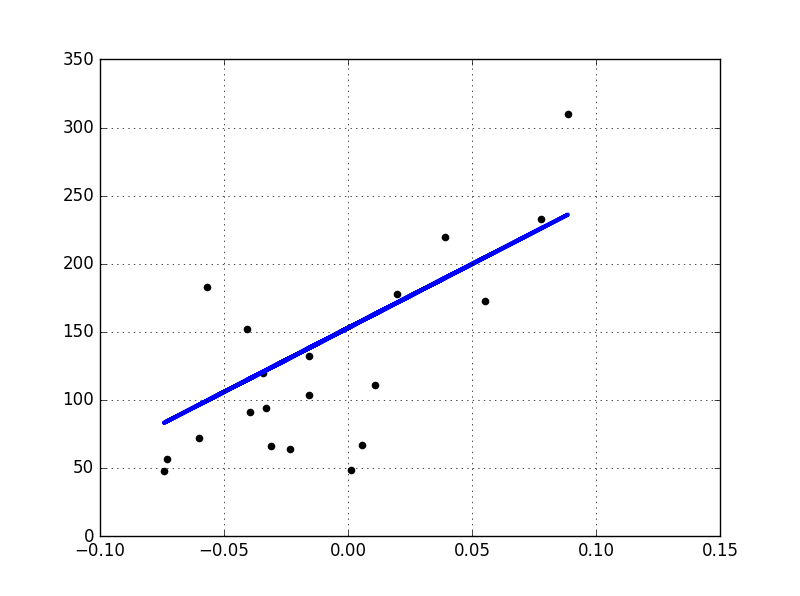最小二乘法（Ordinary Least Squares）
线性回归（Linear Regression）通过寻找合适的

ω={w0,w1,w2,...,wn}
$\omega=\{w_{0},w_{1},w_{2},...,w_{n}\}$，使得观测样本集合

X
$X$（这里观测样本集合X$X$在很多的外文书籍中比如RPML，叫做设计矩阵（design matrix）），和目标输出

y
$y$之间的残差平方和最小(residual sum of squares)，从数学上来说，其的目标问题或者叫做损失函数是：
J(w)=minw∥Xw−y∥2J(w)=\min_{w}\left \| Xw-y \right \|^{2}
最小乘法的证明
对于上面的损失函数而言，

J(w)
$J(w)$显然是非负的，其去最小值的充要条件就是

∥Xw−y∥2
$\left \| Xw-y \right \|^{2}$的倒数为0时的

w
$w$的取值，从矩阵的角度来写这个式子：
∥Xw−y∥2=(Xw−y)T(Xw−y)\left \| Xw-y \right \|^{2}=(Xw-y)^T(Xw-y)
容易得到其关于

w
$w$的导数为：
XT(Xw−y)+XT(Xw−y)=0X^T(Xw-y) + X^T(Xw-y)=0
即：

w=(XTX)−1XTy

w=(X^TX)^{-1}X^Ty

这里，我们将

X+≡(XTX)−1XT
$X^+\equiv(X^TX)^{-1}X^T$定义为X的伪逆矩阵。
这个是一个非常好的结果，它说明使用最小二乘法，从数学上是可以直接通过公式来求解出参数

w
$w$的。

注意：最小二乘法对ω$\omega$的估计，是基于模型中变量之间相互独立的基本假设的，即输入向量

x
$x$中的任意两项xi$x_{i}$和

xj
$x_{j}$之间是相互独立的。如果输入矩阵

X
$X$中存在线性相关或者近似线性相关的列，那么输入矩阵X$X$就会变成或者近似变成奇异矩阵（singular matrix）。这是一种病态矩阵，矩阵中任何一个元素发生一点变动，整个矩阵的行列式的值和逆矩阵都会发生巨大变化。这将导致最小二乘法对观测数据的随机误差极为敏感，进而使得最后的线性模型产生非常大的方差，这个在数学上称为多重共线性（multicollinearity）。在实际数据中，变量之间的多重共线性是一个非常普遍的现象，其产生机理及相关解决方案在“特征选择和评估”中有介绍。
基于基函数的线性回归模型
前面已经提到过，线性回归模型的基本特征就是，模型是参数向量

ω={w0,w1,w2,...,wn}
$\omega=\{w_{0},w_{1},w_{2},...,w_{n}\}$的线性函数，上面的那个例子模型同时也是输入变量

x
$x$的线性函数，这极大地限制了模型的适用性。这里使用基函数（basis function）对上面的线性模型进行拓展，即：线性回归模型是一组输入变量x$x$的非线性基函数的线性组合，在数学上其形式如下：

y(x,w)=w0+∑j=1Mωjϕj(x)

y(x,w)=w_{0}+\sum_{j=1}^{M}\omega_{j}\phi_{j}(x)

这里

ϕj(x)
$\phi_{j}(x)$就是前面提到的基函数，总共的基函数的数目为

M
$M$个，如果定义ϕ0(x)=1$\phi_{0}(x)=1$的话，那个上面的式子就可以简单的表示为：

y(x,w)=∑j=0Mωjϕj(x)=wTϕ(x)

y(x,w)=\sum_{j=0}^{M}\omega_{j}\phi_{j}(x)=w^T\phi(x)

w=(w0,w1,w2,...,wM)

w=(w_{0},w_{1},w_{2},...,w_{M})

ϕ=(ϕ0,ϕ1,ϕ2,...,ϕM)

\phi=(\phi_{0},\phi_{1},\phi_{2},...,\phi_{M})

在“特征选择和评估”中，实际拿到手的数据，由于各种原因，往往不能直接应用到机器学习算法中，需要对原始数据做一些预处理或者是特征提取的操作，而那些从原始输入向量

x
$x$中提取出来的特征， 就是这一个个的ϕj(x)$\phi_{j}(x)$.
通过使用非线性的基函数，我们可以使得线性模型

y(x,w)
$y(x,w)$成为输入向量

x
$x$的非线性函数。

下面举一些基于不同基函数的线性模型y(x,w)$y(x,w)$的例子:

ϕj(x)=xj
$\phi_{j}(x)=x_{j}$  这里取

M=n
$M=n$,那么就可以很容易的得到：

y(x,w)=w0+∑j=1Mωjϕj(x)=w0+w1∗x1+w2∗x2+...+wn∗xn

\begin{aligned}
y(x,w) &= w_{0}+\sum_{j=1}^{M}\omega_{j}\phi_{j}(x)\\
&=w_{0} + w_{1}*x_{1}+ w_{2}*x_{2}+...+ w_{n}*x_{n}
\end{aligned}

ϕj(x)=xj
$\phi_{j}(x)=x^{j}$  这里就是典型的多项式曲线拟合（Polynomial Curve Fitting），其中

M
$M$是输入变量x$x$的最高次数，同时也是模型的复杂度。多项式曲线拟合的一个缺陷就是这个模型是一个输入变量的全局函数，这使得对输入空间的一个区间的改动会影响到其他的区间，这个问题可以通过将输入空间切分成不同的区域，对不同的区域各自在各自的区域中做曲线拟合来解决，这个方法就是经典的样条函数（spline function）。笔记中有专门的一节详细的论述了多项式曲线拟合相关的特性。

y(x,w)=w0+∑j=1Mωjϕj(x)=∑j=0Mωjxj

\begin{aligned}
y(x,w) &= w_{0}+\sum_{j=1}^{M}\omega_{j}\phi_{j}(x)\\
&= \sum_{j=0}^M\omega_{j}x^j
\end{aligned}

ϕj(x)=exp{−(x−μj)22s2}
$\phi_{j}(x)=exp\{-\frac{(x-\mu_{j})^2}{2s^2}\}$  这里

μj
$\mu_{j}$表示的是该基函数在输入空间中的位置，参数

s
$s$控制的是基函数的空间尺度，这个基函数往往会被称为高斯基函数（Gaussian basis function）。需要强调一点，这里的高斯函数并不是输入空间的概率分布，其并没有概率意义。由于每个基函数前面都有一个比例系数ωj$\omega_{j}$，所以，对参数的归一化（coefficient normalization）并不是必需的。

y(x,w)=w0+∑j=1Mωjϕj(x)=w0+∑j=1Mωjexp{−(x−μj)22s2}

\begin{aligned}
y(x,w) &= w_{0}+\sum_{j=1}^{M}\omega_{j}\phi_{j}(x)\\
&=w_{0} + \sum_{j=1}^{M}\omega_{j}exp\{-\frac{(x-\mu_{j})^2}{2s^2}\}
\end{aligned}

ϕj(x)=σ(x−μjs)
$\phi_{j}(x)=\sigma(\frac{x-\mu_j}{s})$  这个基函数叫做

sigmoid
$sigmoid$函数，其定义为：

σ(a)=11+exp(−a)

\sigma(a)=\frac{1}{1+exp(-a)}  与这个函数拥有相同效果的一个函数叫做

tach
$tach$函数，其定义为：

tanh(a)=2σ(a)−1

tanh(a)=2\sigma(a)-1 在神经网络中，这两个基函数有着非常广泛的应用。其实从根本上来讲，神经网络的每一层，也是一些基函数的线性组合，神经网络之所以能处理非线性问题，其根本也是由于采用了合适的非线性基函数。

y(x,w)=w0+∑j=1Mωjϕj(x)=w0+∑j=1Mωj11+exp(−a)

\begin{aligned}
y(x,w) &= w_{0}+\sum_{j=1}^{M}\omega_{j}\phi_{j}(x)\\
&=w_{0} + \sum_{j=1}^{M}\omega_{j}\frac{1}{1+exp(-a)}
\end{aligned}

线性回归的在线学习算法
在《多项式回归》中，介绍了线性模型的极大似然估计解释：对于训练数据

{X,t}
$\{X,t\}$而言，可以使用极大似然估计来计算参数

w
$w$和σ2$\sigma^2$，其中

w
$w$ 是 y(xn,w)$y(x_{n},w)$ 的参数；而

σ2
$\sigma^2$ 是噪声的方差：

p(t|X,w,σ2)=∏n=1NN(tn|y(xn,w),σ2)

p(t|X,w,\sigma^2)=\prod_{n=1}^NN(t_{n}|y(x_{n},w),\sigma^2)

像OLS算法这种，一次性就将训练数据集中的

N
$N$个数据全部都用于p(t|X,w,σ2)$p(t|X,w,\sigma^2)$的估计中，然后通过求导得到参数的估计结果。这种算法的模型，称为批处理技术（Batch techniques），在Andrew Ng的机器学习课程视频中，对这个有说明。其主要缺点就在于，面对大的数据集的时候，其计算成本会非常的大。
当数据集特别大的时候，这种批处理技术就不再适用，就有一种称为序列学习算法（Sequential learning），也叫作在线算法（on-line algorithms）的算法得到了广泛的应用。
说得简单一点，序列学习算法就是将数据的样本点，看成在时间上有序的，即，数据集合不是一次性得到全部的数据，而是一个样本一个样本采集得到的。那么，在训练算法的时候，也就可以一个样本一个样本的输入的训练算法的中，利用新的样本不断的改进模型，进而达到学习的目的。
梯度下降法
有前面可以知道，线性回归的损失函数为:

J(w)=minw∥Xw−y∥2

J(w)=\min_{w}\left \| Xw-y \right \|^{2}

其关于参数

w
$w$梯度为：

∇J(w)=2XT(Xw−y)\nabla J(w)=2X^T(Xw-y)
梯度下降法就是：

wk=wk−1−ρk∇J(w)

w_{k} = w_{k-1}- \rho_{k} \nabla J(w)

通过迭代，求得

wk
$w_{k}$
随机梯度下降法
随机梯度下降法，就是这里要说的在线学习算法，它不像上面的梯度下降法，每次迭代都使用了全部的数据集，这里每次迭代仅仅使用一个样本：

wk=wk−1−ρkxk(xTkwk−1−yk)

w_{k} = w_{k-1}- \rho_{k} x_{k}(x_{k}^Tw_{k-1} - y_{k})

这里需要说明的是，

ρk
$\rho_{k}$ 必须满足两个条件：

∑k=1∞ρk→∞∑k=1∞ρ2k<∞

\sum_{k=1}^{\infty}\rho_{k}\to\infty \\
\sum_{k=1}^{\infty}\rho_{k}^2 < \infty

这个过程，其实是根据新的输入样本，对

wk
$w_{k}$的一个不断的修正的过程，一般来说，我们会把

ρk
$\rho_{k}$后面的式子称为修正量。
折两个条件，保证了在迭代过程中估计的修正值，会趋近于零。因此，对于足够大的

k
<script type="math/tex" id="MathJax-Element-182">k</script>而言，迭代会突然停止，但是，由于有第一个条件的存在，这个停止不会发生的太早，并且不会再离结果非常远的时候，就停止了迭代。上面的第二个条件保证了，对于变量的随机性噪声，噪声的累积保持有限。
展开全文• 线性回归1.线性回归1.1 线性模型(Linear Model)1.2 损失函数(Loss Function):Square Loss1.3 求解1.3.1 最小二乘法(Least Square Method)1.3.2 梯度下降法(Gradient Descent)1.4 正则化(Regularization) 1.线性回归 ...
1.线性回归
1.1 线性模型(Linear Model)
给定数据集

D

=

{

(

x

1

,

y

1

)

,

(

x

2

,

y

2

)

,

(

x

3

,

y

3

)

,

.

.

.

,

(

x

m

,

y

m

)

}

D=\left \{ \left (x_{1}\mathbf{},y_{1} \right ),\left ( x_{2},y_{2} \right ),\left (x_{3},y_{3} \right ),...,\left (x_{m},y_{m} \right ) \right \}

，其中

x

i

x_{i}

有

d

d

个特征，表示为

x

i

=

(

x

i

1

;

x

i

1

;

.

.

.

;

x

i

d

)

x_{i}=\left ( x_{i1}; x_{i1};...;x_{id}\right )

,线性回归模型是通过

d

d

个特征的线性组合对

y

y

值进行拟合，即

f

(

x

i

)

=

w

T

x

i

+

b

f(x_{i})=w^{T}x_{i}+b

,使得

f

(

x

i

)

≃

y

i

f(x_{i})\simeq y_{i}

1.2 损失函数(Loss Function):Square Loss
定义：

L

(

f

(

x

)

,

y

)

=

(

f

(

x

)

−

y

)

2

L(f(x),y)=(f(x)-y)^2

为什么使用square loss作为损失函数：

(1)记误差

ε

=

y

i

−

y

i

^

\varepsilon=y_{i}-\hat{y_{i}}

,假设误差独立同分布，根据中心极限定理，

ε

∼

(

μ

,

σ

2

)

\varepsilon\sim(\mu,\sigma^2)

,得到

f

(

ε

)

=

1

σ

2

π

e

x

p

(

−

(

ε

−

μ

)

2

2

σ

2

)

f(\varepsilon)=\frac{1}{\sigma\sqrt{2\pi}}exp(-\frac{(\varepsilon-\mu)^2}{2\sigma^2})

,求

μ

\mu

和

σ

2

\sigma^2

的极大似然估计，

L

(

μ

,

σ

2

)

=

∏

i

=

1

m

1

σ

2

π

e

x

p

(

−

(

ε

−

μ

)

2

2

σ

2

)

L(\mu,\sigma^2)=\prod_{i=1}^{m}\frac{1}{\sigma\sqrt{2\pi}}exp(-\frac{(\varepsilon-\mu)^2}{2\sigma^2})

,等式两边取对数，得到，

l

o

g

L

(

μ

,

σ

2

)

=

−

m

2

l

o

g

2

π

−

m

2

l

o

g

σ

2

−

(

ε

−

μ

)

2

2

σ

2

logL(\mu,\sigma^2)=-\frac{m}{2}log2\pi-\frac{m}{2}log\sigma^2-\frac{(\varepsilon-\mu)^2}{2\sigma^2}

,对

μ

\mu

和

σ

2

\sigma^2

求偏导，得到

∂

L

∂

μ

=

1

σ

2

(

ε

−

μ

)

\frac{\partial{L}}{\partial{\mu}}=\frac{1}{\sigma^2}(\varepsilon-\mu)

,

∂

L

∂

σ

2

=

m

2

σ

2

+

(

ε

−

μ

)

2

2

σ

4

\frac{\partial{L}}{\partial{\sigma^2}}=\frac{m}{2\sigma^2}+\frac{(\varepsilon-\mu)^2}{2\sigma^4}

, 令

∂

L

∂

μ

=

∂

L

∂

σ

2

=

0

\frac{\partial{L}}{\partial{\mu}}=\frac{\partial{L}}{\partial{\sigma^2}}=0

，得到：

μ

=

1

m

∑

i

=

1

m

ε

i

=

1

m

∑

i

=

1

m

(

y

i

−

y

i

^

)

\mu=\frac{1}{m}\sum_{i=1}^{m}\varepsilon_{i}=\frac{1}{m}\sum_{i=1}^{m}(y_{i}-\hat{y_{i}})

趋近于0或越小越好 ,

σ

2

=

1

m

∑

i

=

1

m

(

ε

i

−

μ

)

2

=

1

m

∑

i

=

1

m

(

y

i

−

y

i

^

−

μ

)

2

≈

1

m

∑

i

=

1

m

(

y

i

−

y

i

^

)

2

\sigma^2=\frac{1}{m}\sum_{i=1}^{m}(\varepsilon_{i}-\mu)^2=\frac{1}{m}\sum_{i=1}^{m}(y_{i}-\hat{y_{i}}-\mu)^2\approx\frac{1}{m}\sum_{i=1}^{m}(y_{i}-\hat{y_{i}})^2

趋近于0或越小越好,与最前面构建的平方形式损失函数本质上是等价的。 (2)误差的平方形式是正数。这样正的误差和负的误差不会相互抵消。 (3)与采用绝对值形式的比较：平方形式对大误差的惩罚大于小误差；平方形式对数学运算也更友好，绝对值形式在求导使需要分段求导。

定义代价函数(cost function):

J

(

w

,

b

)

=

1

2

m

∑

i

=

1

m

(

f

(

x

i

)

−

y

i

)

2

J(w,b)=\frac{1}{2m}\sum_{i=1}^{m}(f(x_{i})-y_{i})^2

1.3 求解
引入向量形式

θ

=

(

w

,

b

)

,

X

=

[

x

11

x

12

⋯

x

1

d

1

x

21

x

22

⋯

x

2

d

1

⋮

⋮

⋱

⋮

⋮

x

m

1

x

m

2

⋯

x

m

d

1

]

=

[

x

1

T

1

x

2

T

1

⋮

⋮

x

m

T

1

]

\theta=(w,b),X=\begin{bmatrix} x_{11} &amp; x_{12} &amp; \cdots &amp; x_{1d}&amp;1 \\ x_{21} &amp; x_{22} &amp; \cdots &amp; x_{2d}&amp;1 \\ \vdots &amp;\vdots &amp; \ddots &amp; \vdots&amp;\vdots\\ x_{m1} &amp; x_{m2} &amp; \cdots &amp; x_{md}&amp;1 \end{bmatrix}=\begin{bmatrix} x_{1}^{T}&amp;1 \\ x_{2}^{T}&amp;1 \\ \vdots &amp;\vdots \\ x_{m}^{T} &amp; 1 \end{bmatrix}

, 则

J

(

θ

)

=

1

2

∑

i

=

1

m

(

h

θ

(

x

i

)

−

y

i

)

2

=

1

2

(

X

θ

−

y

)

T

(

X

θ

−

y

)

J(\theta)=\frac{1}{2}\sum_{i=1}^{m}(h_{\theta}(x_{i})-y_{i})^2=\frac{1}{2}(X\theta-y)^T(X\theta-y)

1.3.1 最小二乘法(Least Square Method)
在线性回归中，最小二乘法就是试图找到一条直线，使所有样本到直线上的欧式距离之和最小。

求梯度 \$\nabla_{\theta}J(\theta)

=

∇

θ

(

1

2

(

θ

T

X

T

−

y

T

)

(

X

θ

−

y

)

)

=\nabla_{\theta}\left(\frac{1}{2}(\theta^TX^T-y^T)(X\theta-y)\right)

=

∇

θ

(

1

2

(

θ

T

X

T

X

θ

−

θ

T

X

T

y

−

y

T

X

θ

+

y

T

y

)

)

=\nabla_{\theta}\left(\frac{1}{2}(\theta^TX^TX\theta-\theta^TX^Ty-y^TX\theta+y^Ty)\right)

=

1

2

(

2

X

T

X

θ

−

X

T

y

−

(

y

T

X

)

T

)

=\frac{1}{2}\left(2X^TX\theta-X^Ty-(y^TX)^T\right)

=

X

T

X

θ

−

X

T

y

=X^TX\theta-X^Ty

令上式等于0，求驻点，则有

θ

=

(

X

T

X

)

−

1

X

T

y

\theta=(X^TX)^{-1}X^Ty

, 则令

x

i

^

=

(

x

i

;

1

)

\hat{x_{i}}=(x_{i};1)

线性回归模型为

f

(

X

)

=

x

i

^

T

(

X

T

X

)

−

1

X

T

y

f(X)=\hat{x_{i}}^T(X^TX)^{-1}X^Ty

1.初始化

θ

\theta

2.沿着负梯度方向迭代，得到

θ

=

θ

−

α

⋅

∂

J

∂

θ

\theta=\theta-\alpha\cdot\frac{\partial J}{\partial \theta}

，使得

J

(

θ

)

J(\theta)

更小，

α

\alpha

为学习率(learning rate)(也称为步长)， 偏导计算

∂

J

∂

θ

j

=

(

h

θ

(

x

)

−

y

)

⋅

∂

∂

θ

j

(

∑

i

=

1

m

θ

i

x

i

−

y

)

=

(

h

θ

(

x

)

−

y

)

x

j

\frac{\partial J}{\partial \theta_{j}}=(h_{\theta}(x)-y)\cdot\frac{\partial }{\partial \theta_j}(\sum_{i=1}^{m}\theta_{i}x_{i}-y)=(h_{\theta}(x)-y)x_j

3.直至下降到无法下降时，停止计算

1.4 正则化(Regularization)
现实往往其变量数目超过样本数，

X

T

X

X^TX

不满秩，导致存在多个

θ

^

\hat{\theta}

,使得

J

(

θ

)

J(\theta)

最小化。此时，选择哪个解作为输出，由学习算法的归纳偏好决定，常见的做法是引入正则化项，通过减少参数

θ

\theta

的值，使得模型变得简单，避免过拟合。 正则化线性回归(regularized linear regression)的代价函数（cost function）为：
L1-norm(LASSO): 假设参数的先验分布是Laplace分布，

J

(

θ

)

=

1

2

∑

i

=

1

m

(

h

θ

(

x

i

)

−

y

i

)

2

+

λ

∑

i

=

1

m

∣

θ

j

∣

J(\theta)=\frac{1}{2}\sum_{i=1}^{m}(h_{\theta}(x_{i})-y_{i})^2+\lambda\sum_{i=1}^{m}|\theta_j|

L1范数可以实现稀疏化，而本质上对

θ

\theta

进行稀疏约束，即希望

θ

\theta

非零分量尽可能少，常用L0范数，但L0范数不连续，很难优化求解（NP问题）。而在求解向量足够稀疏的情况下，L0范数优化问题等价于L1范数优化问题，即各向量分量绝对值之和。 L1的优势在于通过稀疏化可用于特征选择，但也因此导致局部特征没有完全体现出来。L2-norm(Ridge): 假设参数的先验分布是Gaussian 分布，

J

(

θ

)

=

1

2

∑

i

=

1

m

(

h

θ

(

x

i

)

−

y

i

)

2

+

λ

∑

i

=

1

m

θ

j

2

J(\theta)=\frac{1}{2}\sum_{i=1}^{m}(h_{\theta}(x_{i})-y_{i})^2+\lambda\sum_{i=1}^{m}\theta_j^2

为了解决L1没有保留局部特征的问题，引入L2范数替代L1范数。L2范数使得

θ

\theta

趋向于0，

θ

\theta

越小使得模型的复杂度降低。Elastic Net:

J

(

θ

)

=

1

2

∑

i

=

1

m

(

h

θ

(

x

i

)

−

y

i

)

2

+

λ

(

ρ

⋅

∑

i

=

1

m

∣

θ

j

∣

+

(

1

−

ρ

)

∑

i

=

1

m

θ

j

2

)

J(\theta)=\frac{1}{2}\sum_{i=1}^{m}(h_{\theta}(x_{i})-y_{i})^2+\lambda\left(\rho\cdot\sum_{i=1}^{m}|\theta_j|+(1-\rho)\sum_{i=1}^{m}\theta_j^2\right)

其中，L1正则项产生稀疏模型，L2正则项消除L1正则项中选择变量个数的限制（即稀疏性）。 Elastic Net在高度相关变量的情况下，会产生群体效应；选择变量的数目没有限制；可以承受双重收缩。
2.广义线性回归(Generalized linear model，GLM)
2.1 定义
2.1.1 指数族分布
给定随机变量

Y

Y

，观测值为

y

y

,服从指数型分布，即有概率分布函数：

p

(

y

;

η

)

=

b

(

y

)

e

x

p

(

η

T

T

(

y

)

−

a

(

η

)

)

p(y;η)=b(y)exp(η^TT(y)−a(η))

,其中

η

η

为自然参数(bature parameter)，

T

(

y

)

T(y)

是充分统计量(sufficient statistic)。常见的指数族分布：伯努利分布、高斯分布、多项式分布、泊松分布、指数分布、伽马分布、贝塔分布、狄利克雷分布、维希特分布……
2.1.2 广义线性模型
GLM必须满足以下3个假设：

y

∣

x

,

θ

∼

E

x

p

o

n

e

n

t

i

a

l

F

a

m

i

l

y

(

η

)

y|x,\theta\sim ExponentialFamily(η)

预测的值

h

θ

(

x

)

=

E

[

T

(

y

)

∣

x

]

h_{\theta}(x)=E[T(y)|x]

,通常

T

(

y

)

=

y

T(y)=y

假设

E

(

Y

)

=

μ

E(Y)=\mu

,且

μ

\mu

与

η

\eta

由如下关系:

g

(

μ

)

=

η

g(\mu)=\eta

,则

μ

=

g

−

1

(

η

)

\mu=g^{-1}(\eta)

,

g

(

μ

)

g(\mu)

η

=

θ

T

x

η=\theta^Tx

2.2 逻辑回归(Logistic Regression)
2.2.1 定义

y

∼

B

(

1

,

φ

)

y\sim B(1,\varphi)

F

(

y

)

=

φ

y

(

1

−

φ

)

(

1

−

y

)

F(y)=\varphi^y(1-\varphi)^{(1-y)}

=

e

x

p

(

y

l

o

g

φ

+

(

1

−

y

)

l

o

g

(

1

−

φ

)

)

=exp\left(ylog\varphi+(1-y)log(1-\varphi)\right)

=

e

x

p

(

y

l

o

g

φ

1

−

φ

+

l

o

g

(

1

−

φ

)

)

=exp\left(ylog\frac{\varphi}{1-\varphi}+log(1-\varphi)\right)

由指数族分布可知，

b

(

y

)

=

1

,

η

=

l

o

g

φ

1

−

φ

b(y)=1,η=log\frac{\varphi}{1-\varphi}

(称为logit),

T

(

y

)

=

y

,

a

(

η

)

=

−

l

o

g

(

1

−

φ

)

T(y)=y,a(η)=-log(1-\varphi)

得到，

φ

=

1

1

+

e

−

η

\varphi=\frac{1}{1+e^{-η}}

(sigmoid函数)

h

θ

(

x

)

=

E

[

T

(

y

)

∣

x

]

=

φ

h_{\theta}(x)=E[T(y)|x]=\varphi

η

=

θ

T

x

η=\theta^Tx

由此得到

h

θ

(

x

)

=

1

1

+

e

θ

T

x

h_{\theta}(x)=\frac{1}{1+e^{\theta^Tx}}

2.2.2 损失函数(Loss Function)
loss function：对数损失, 即对数似然损失(Log-likelihood Loss), 也称逻辑斯谛回归损失(Logistic Loss)

L

(

θ

)

=

∏

i

=

1

m

P

(

y

∣

x

;

θ

)

L(\theta)=\prod_{i=1}^{m}P(y|x;\theta)

=

∏

i

=

1

m

h

θ

(

x

i

)

y

i

(

1

−

h

θ

(

x

i

)

)

(

1

−

y

i

)

=\prod_{i=1}^{m}h_{\theta}(x_i)^{y_i}(1-h_{\theta}(x_i))^{(1-y_i)}

l

o

g

L

(

θ

)

logL(\theta)

=

∑

i

=

1

m

l

o

g

(

h

θ

(

x

i

)

y

i

(

1

−

h

θ

(

x

i

)

)

(

1

−

y

i

)

)

=\sum_{i=1}^{m}log(h_{\theta}(x_i)^{y_i}(1-h_{\theta}(x_i))^{(1-y_i)})

=

∑

i

=

1

m

[

y

i

l

o

g

h

θ

(

x

i

)

+

(

1

−

y

i

)

l

o

g

(

1

−

h

θ

(

x

i

)

)

]

=\sum_{i=1}^{m}[y_ilogh_{\theta}(x_i)+(1-y_i)log(1-h_{\theta}(x_i))]

cost function通过最小化负的对数似然函数得到：

J

(

θ

)

=

−

1

m

∑

i

=

1

m

[

y

i

l

o

g

h

θ

(

x

i

)

+

(

1

−

y

i

)

l

o

g

(

1

−

h

θ

(

x

i

)

)

]

J(\theta)=-\frac{1}{m}\sum_{i=1}^{m}[y_ilogh_{\theta}(x_i)+(1-y_i)log(1-h_{\theta}(x_i))]

为什么使用logitic loss而不是square loss
极大似然法求解得到的结果与对数似然函数的结果是等价的（推导如上）  逻辑回归的平方损失不是一个凸函数，不容易求解，得到的解是局部最优解。 对数损失是一个凸函数，且是关于

θ

\theta

的高阶连续可导的凸函数（证略，多元函数的海赛矩阵半正定是其为凸函数的充分必要条件）
2.2.3 求解
梯度下降法：同1.3.2
2.2.4 逻辑回归和线性回归的区别和联系
两者都是属于广义线性回归的特殊情况  线性回归是假设y服从高斯分布，逻辑回归假设y服从伯努利分布  线性回归的损失函数是square loss，而逻辑回归的损失函数是logistic loss
2.2.5 优缺点

优点： (1).模型的可解释性强，可以通过参数值看到特征对结果的影响（该值并不能代表特征的重要性）。 (2).模型的训练速度较快 (3).既可以得到分类结果也可以得到类别的概率值 缺点： (1).模型的准确性不高 (2).数据不平衡时，对正负样本的区分能力差 (3).对模型中自变量多重共线性较为敏感 (4).不适合处理非线性数据,只能处理二分类的问题。 (5).sigmoid函数两端斜率小，模型输出的概率值变化小，中间段斜率大，概率变化大。这导致特征某些区间的数值变化对概率的影响较大。

现实场景中，应用逻辑回归往往先进行特征归一化、特征离散化和交叉化处理
展开全文机器学习
• 一般线性模型和线性回归模型Interpretability is one of the biggest challenges in machine learning. A model has more interpretability than another one if its decisions are easier for a human to ...

一般线性模型和线性回归模型

Interpretability is one of the biggest challenges in machine learning. A model has more interpretability than another one if its decisions are easier for a human to comprehend. Some models are so complex and are internally structured in such a way that it’s almost impossible to understand how they reached their final results. These black boxes seem to break the association between raw data and final output, since several processes happen in between.
可解释性是机器学习中最大的挑战之一。 如果一个模型的决策更容易让人理解，那么它的解释性就会比另一个模型高。 有些模型是如此复杂，并且内部结构如此复杂，以至于几乎无法理解它们是如何达到最终结果的。 这些黑匣子似乎打破了原始数据和最终输出之间的关联，因为它们之间发生了多个过程。
But in the universe of machine learning algorithms, some models are more transparent than others. Decision Trees are definitely one of them, and Linear Regression models are another one. Their simplicity and straightforward approach turns them into an ideal tool to approach different problems. Let’s see how.
但是在机器学习算法领域，某些模型比其他模型更透明。 决策树绝对是其中之一，而线性回归模型又是其中之一。 它们的简单和直接的方法使它们成为解决不同问题的理想工具。 让我们看看如何。
You can use Linear Regression models to analyze how salaries in a given place depend on features like experience, level of education, role, city they work in, and so on. Similarly, you can analyze if real estate prices depend on factors such as their areas, numbers of bedrooms, or distances to the city center.
您可以使用线性回归模型来分析给定地点的薪水如何取决于经验，学历，职位，所工作的城市等特征。 同样，您可以分析房地产价格是否取决于面积，卧室数量或距市中心的距离等因素。
In this post, I’ll focus on Linear Regression models that examine the linear relationship between a dependent variable and one (Simple Linear Regression) or more (Multiple Linear Regression) independent variables.
在本文中，我将重点介绍线性回归模型，该模型研究因变量与一个(简单线性回归)或多个(多个线性回归) 自变量之间的线性关系。
简单线性回归(SLR) (Simple Linear Regression (SLR))
Is the simplest form of Linear Regression used when there is a single input variable (predictor) for the output variable (target):
当输出变量(目标)只有一个输入变量(预测变量)时，是使用线性回归的最简单形式：
The input or predictor variable is the variable that helps predict the value of the output variable. It is commonly referred to as X. 输入或预测变量是有助于预测输出变量值的变量。 通常称为X。 The output or target variable is the variable that we want to predict. It is commonly referred to as y. 输出或目标变量是我们要预测的变量。 通常称为y 。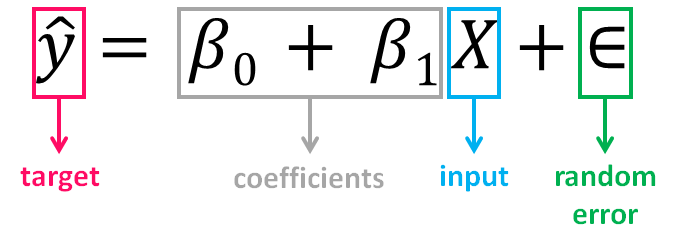The value of β0, also called the intercept, shows the point where the estimated regression line crosses the y axis, while the value of β1 determines the slope of the estimated regression line. The random error describes the random component of the linear relationship between the dependent and independent variable (the disturbance of the model, the part of y that X is unable to explain). The true regression model is usually never known (since we are not able to capture all the effects that impact the dependent variable), and therefore the value of the random error term corresponding to observed data points remains unknown. However, the regression model can be estimated by calculating the parameters of the model for an observed data set.
β0的值( 也称为截距 )显示估算的回归​​线与y轴交叉的点，而β1的值确定估算的回归​​线的斜率 。 随机误差描述了因变量和自变量之间线性关系的随机成分(模型的扰动， X无法解释的y部分)。 真正的回归模型通常是未知的(因为我们无法捕获影响因变量的所有影响)，因此与观察到的数据点相对应的随机误差项的值仍然未知。 但是，可以通过为观察到的数据集计算模型的参数来估计回归模型。
The idea behind regression is to estimate the parameters β0 and β1 from a sample. If we are able to determine the optimum values of these two parameters, then we will have the line of best fit that we can use to predict the values of y, given the value of X. In other words, we try to fit a line to observe a relationship between the input and output variables and then further use it to predict the output of unseen inputs.
回归背后的想法是从样本中估计参数β0和β1 。 如果我们能够确定这两个参数的最佳值，则在给定X的值的情况下，我们将具有最佳拟合线，可用于预测y的值。 换句话说，我们尝试拟合一条线以观察输入变量和输出变量之间的关系，然后进一步使用它来预测未见输入的输出。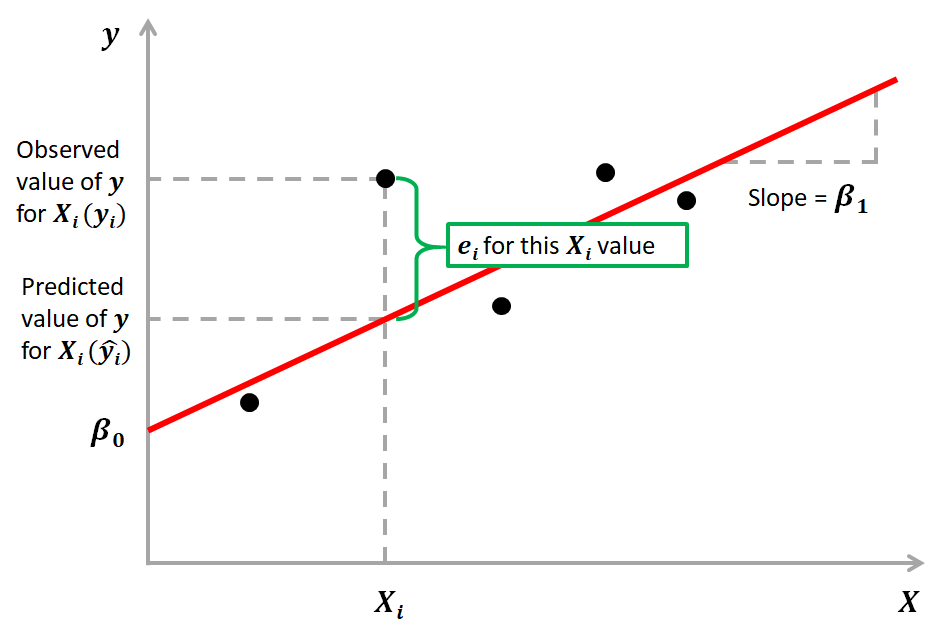How do we estimate β0 and β1? We can use a method called Ordinary Least Squares (OLS). The goal behind this is to minimize the distance from the black dots to the red line as close to zero as possible, which is done by minimizing the squared differences between actual and predicted outcomes.
我们如何估计β0   和β1 ？ 我们可以使用一种称为普通最小二乘(OLS)的方法 。   其背后的目标是使黑点到红线的距离尽可能地接近零，这是通过最小化实际结果与预测结果之间的平方差来实现的。
The difference between actual and predicted values is called residual (e) and can be negative or positive depending on whether the model overpredicted or underpredicted the outcome. Hence, to calculate the net error, adding all the residuals directly can lead to the cancellations of terms and reduction of the net effect. To avoid this, we take the sum of squares of these error terms, which is called the Residual Sum of Squares (RSS).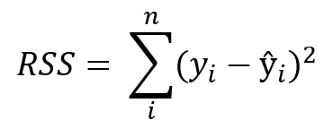The Ordinary Least Squares (OLS) method minimizes the residual sum of squares, and its objective is to fit a regression line that would minimize the distance (measured in quadratic values) from the observed values to the predicted ones (the regression line).
普通最小二乘法(OLS)方法使残差平方和最小化 ，其目的是拟合一条回归线，以使从观测值到预测值的距离(以二次值度量)最小化(回归线)。
多元线性回归(MLR) (Multiple Linear Regression (MLR))
Is the form of Linear Regression used when there are two or more predictors or input variables. Similar to the SLR model described before, it includes additional predictors:
是形式   有两个或多个预测变量或输入变量时使用的线性回归系数。 与之前描述的SLR模型类似，它包含其他预测变量：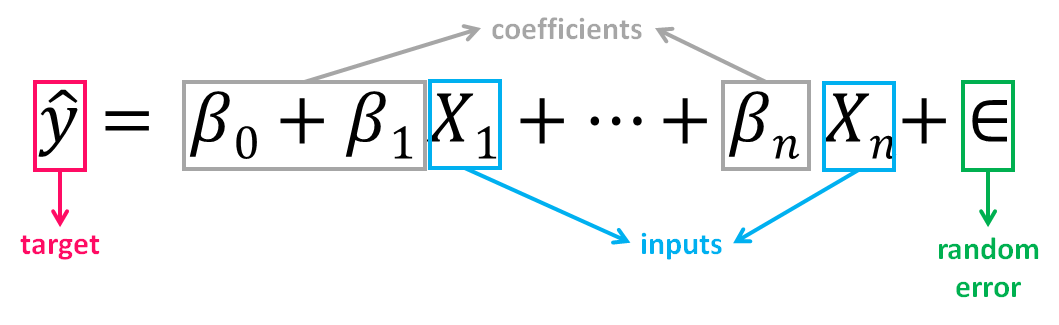Notice that the equation is just an extension of the Simple Linear Regression one, in which each input/ predictor has its corresponding slope coefficient (β). The first β term (β0) is the intercept constant and is the value of y in absence of all predictors (i.e when all X terms are 0).
注意，该方程只是简单线性回归方程的扩展，其中每个输入/预测变量都有其对应的斜率系数(β) 。 第一个β   项(β0)是截距常数，是在没有所有预测变量的情况下(即，当所有X项均为0时)的y值。
As the number of features grows, the complexity of our model increases and it becomes more difficult to visualize, or even comprehend, our data. Because there are more parameters in these models compared to SLR ones, more care is needed when working with them. Adding more terms will inherently improve the fit to the data, but the new terms may not have any real significance. This is dangerous because it can lead to a model that fits that data but doesn’t actually mean anything useful.
随着功能部件数量的增加，我们模型的复杂性也随之增加，并且更加难以可视化甚至理解我们的数据。 由于与SLR相比，这些模型中的参数更多，因此在使用它们时需要格外小心。 添加更多术语会从本质上改善数据的拟合度，但是新术语可能没有任何实际意义。 这很危险，因为它可能会导致模型适合该数据，但实际上并不意味着有用。
一个例子 (An example)
The advertising dataset consists of the sales of a product in 200 different markets, along with advertising budgets for three different media: TV, radio, and newspaper. We’ll use the dataset to predict the amount of sales (dependent variable), based on the TV, radio and newspaper advertising budgets (independent variables).
广告数据集包括产品在200个不同市场中的销售以及三种不同媒体(电视，广播和报纸)的广告预算。 我们将使用数据集根据电视，广播和报纸的广告预算(自变量)来预测销售量(自变量)。
Mathematically, the formula we’ll try solve is:
在数学上，我们将尝试解决的公式是：Finding the values of these constants (β) is what regression model does by minimizing the error function and fitting the best line or hyperplane (depending on the number of input variables). Let’s code.
通过最小化误差函数并拟合最佳直线或超平面(取决于输入变量的数量) ，回归模型可以找到这些常数(β)的值。 让我们编码。
加载数据并描述数据集 (Load data and describe dataset)
您可以在此链接下下载数据集。 在加载数据之前，我们将导入必要的库：
import pandas as pdimport numpy as npimport seaborn as snsimport matplotlib.pyplot as pltfrom sklearn.model_selection import train_test_splitfrom sklearn.linear_model import LinearRegressionfrom sklearn import metricsfrom sklearn.metrics import r2_scoreimport statsmodels.api as sm
现在我们加载数据集：
df = pd.read_csv(“Advertising.csv”)
Let’s understand the dataset and describe it:
让我们了解数据集并对其进行描述：
df.head()We’ll drop the first column (“Unnamed”) since we don’t need it:
我们将删除第一列(“未命名”)，因为我们不需要它：
df = df.drop([‘Unnamed: 0’], axis=1)df.info()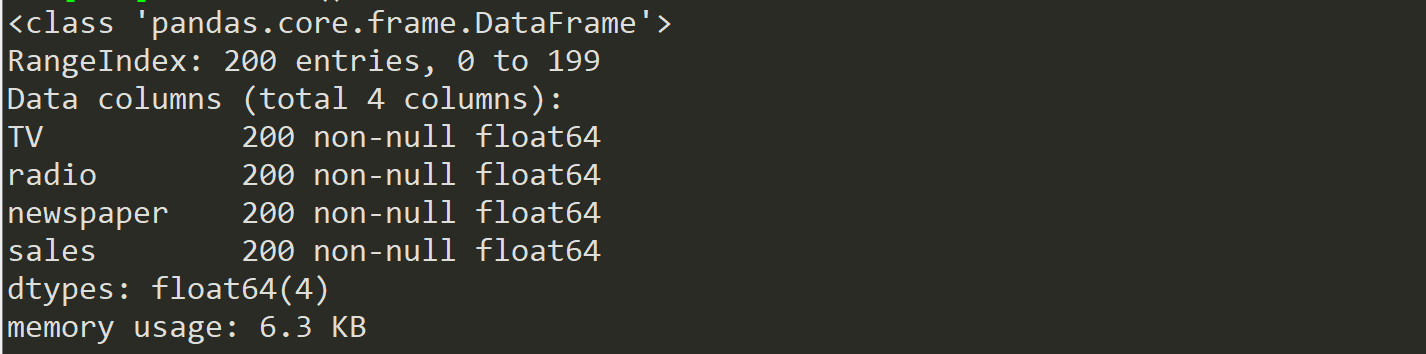Our dataset now contains 4 columns (including the target variable “sales”), 200 registers and no missing values. Let’s visualize the relationship between the independent and target variables.
现在，我们的数据集包含4列(包括目标变量“ sales”)，200个寄存器，并且没有缺失值。 让我们可视化自变量和目标变量之间的关系。
sns.pairplot(df)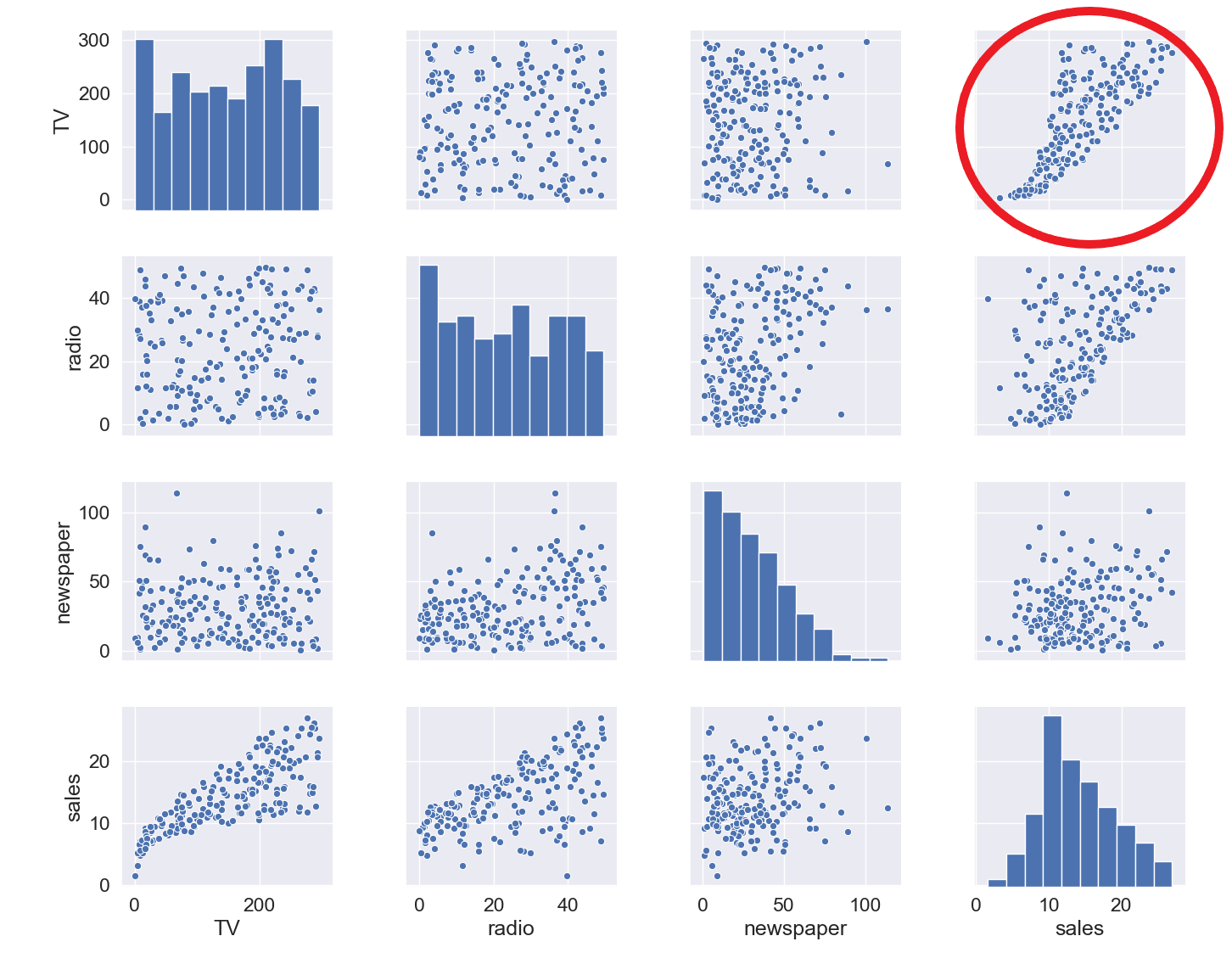The relationship between TV and sales seems to be pretty strong, and while there seems to be some trend between radio and sales, the relationship between newspaper and sales seems to be nonexistent. We can verify that also numerically through a correlation map:
电视与销售之间的关系似乎很牢固，虽然广播与销售之间似乎存在某种趋势，但报纸与销售之间的关系似乎不存在。 我们也可以通过相关图来数值验证：
mask = np.tril(df.corr())sns.heatmap(df.corr(), fmt=’.1g’, annot=True, cmap= ‘cool’, mask=mask)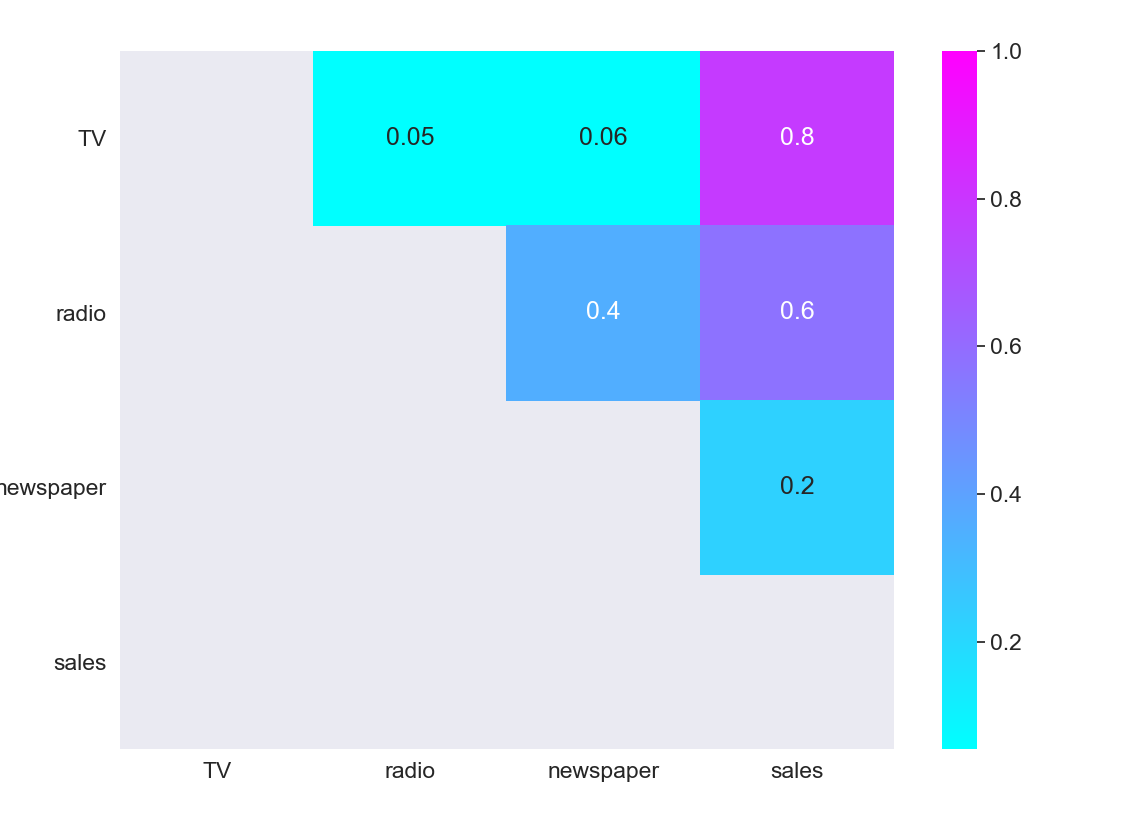As we expected, the strongest positive correlation happens between sales and TV, while the relationship between sales and newspaper is close to 0.
正如我们预期的那样，最强的正相关关系发生在销售和电视之间，而销售和报纸之间的关系接近于0。
选择特征和目标变量 (Select features and target variable)
Next, we divide the variables into two sets: dependent (or target variable “y”) and independents (or feature variables “X”)
接下来，我们将变量分为两组：因变量(或目标变量“ y”)和独立变量(或特征变量“ X”)
X = df.drop([‘sales’], axis=1)y = df[‘sales’]
分割数据集 (Split the dataset)
To understand model performance, dividing the dataset into a training set and a test set is a good strategy. By splitting the dataset into two separate sets, we can train using one set and test the model performance using unseen data on the other one.
为了了解模型的性能，将数据集分为训练集和测试集是一个很好的策略。 通过将数据集分为两个单独的集合，我们可以使用一个集合进行训练，而使用另一集合上的看不见的数据来测试模型的性能。
X_train, X_test, y_train, y_test = train_test_split(X, y, test_size=0.3, random_state=0)
We split our dataset into 70% train and 30% test. The random_state parameter is used for initializing the internal random number generator, which will decide the splitting of data into train and test indices in your case. I set random state = 0 so that you can compare your output over multiple runs of the code using the same parameter.
我们将数据集分为70％训练和30％测试。 random_state参数用于初始化内部随机数生成器，它将根据您的情况决定将数据拆分为训练索引和测试索引。 我将随机状态设置为0，以便您可以使用同一参数在多个代码运行中比较输出。
print(X_train.shape,y_train.shape,X_test.shape,y_test.shape)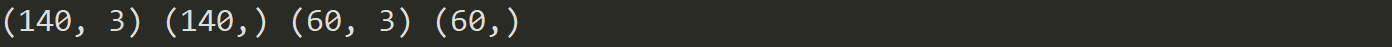By printing the shape of the splitted sets, we see that we created:
通过打印分割集的形状，我们看到我们创建了：
2 datasets of 140 registers each (70% of total registers), one with 3 independent variables and one with just the target variable, that will be used for training and producing the linear regression model. 2个数据集，每个140个寄存器(占总寄存器的70％)，一个包含3个独立变量，一个仅包含目标变量的数据集，将用于训练和生成线性回归模型。 2 datasets of 60 registers each (30% of total registers), one with 3 independent variables and one with just the target variable, that will be used for testing the performance of the linear regression model. 2个数据集，每个60个寄存器(占总寄存器的30％)，一个具有3个独立变量的数据集，一个仅具有目标变量的数据集，这些数据集将用于测试线性回归模型的性能。
建立模型 (Build model)
Building the model is as simple as:
建立模型非常简单：
mlr = LinearRegression()
火车模型 (Train model)
Fitting your model to the training data represents the training part of the modelling process. After it is trained, the model can be used to make predictions, with a predict method call:
使模型适合训练数据代表了建模过程中的训练部分。 训练后，可以使用预测方法调用该模型来进行预测：
mlr.fit(X_train, y_train)
Let’s see the output of the model after being trained, and take a look at the value of β0 (the intercept):
让我们看一下训练后模型的输出，并看一下β0的值(截距)：
mlr.intercept_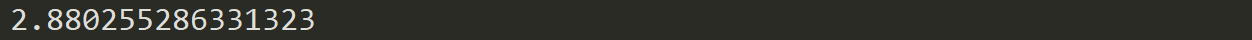We can also print the values of the coefficients (β):
我们还可以打印系数(β)的值：
coeff_df = pd.DataFrame(mlr.coef_, X.columns, columns =[‘Coefficient’])coeff_dfThis way we can now estimate the value of “sales” based on different budget values for TV, radio and newspaper:
这样，我们现在可以根据电视，广播和报纸的不同预算值来估算“销售”的价值：For example, if we determine a budget value of 50 for TV, 30 for radio and 10 for newspaper, the estimated value of “sales” will be:
例如，如果我们确定电视的预算值为50，广播的预算值为30，报纸的预算值为10，则“销售”的估算值将为：
example = [50, 30, 10]output = mlr.intercept_ + sum(example*mlr.coef_)output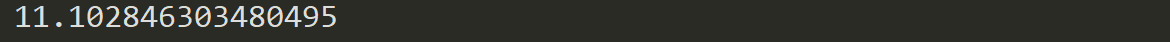测试模型 (Test model)
A test dataset is a dataset that is independent of the training dataset. This test dataset is the unseen data set for your model which will help you have a better view of its ability to generalize:
测试数据集是独立于训练数据集的数据集。 该测试数据集是您模型的看不见的数据集，有助于您更好地了解其概括能力：
y_pred = mlr.predict(X_test)
评估表现 (Evaluate Performance)
The quality of a model is related to how well its predictions match up against the actual values of the testing dataset:
模型的质量与预测与测试数据集的实际值的匹配程度有关：
print(‘Mean Absolute Error:’, metrics.mean_absolute_error(y_test, y_pred))print(‘Mean Squared Error:’, metrics.mean_squared_error(y_test, y_pred))print(‘Root Mean Squared Error:’, np.sqrt(metrics.mean_squared_error(y_test, y_pred)))print(‘R Squared Score is:’, r2_score(y_test, y_pred))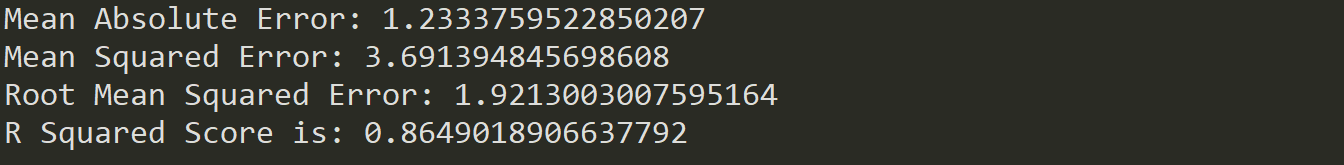After validating our model against the testing set, we get an R² of 0.86 which seems like a pretty decent performance score. But although a higher R² indicates a better fit for the model, it’s not always the case that a high measure is a positive thing. We’ll see below some ways to interpret and improve our regression models.
在根据测试集验证我们的模型后，我们得到的R²为0.86，这似乎是相当不错的性能得分。 但是，尽管较高的R²表示更适合该模型，但并非总是如此。 我们将在下面看到一些解释和改进回归模型的方法。
如何解释和改善您的模型？ (How to interpret and improve your model?)
OK, we created our model, and now what? Let’s take a look at the model statistics over the training data to get some answers:
好的，我们创建了模型，现在呢？ 让我们看一下训练数据上的模型统计信息，以获得一些答案：
X2 = sm.add_constant(X_train)model_stats = sm.OLS(y_train.values.reshape(-1,1), X2).fit()model_stats.summary()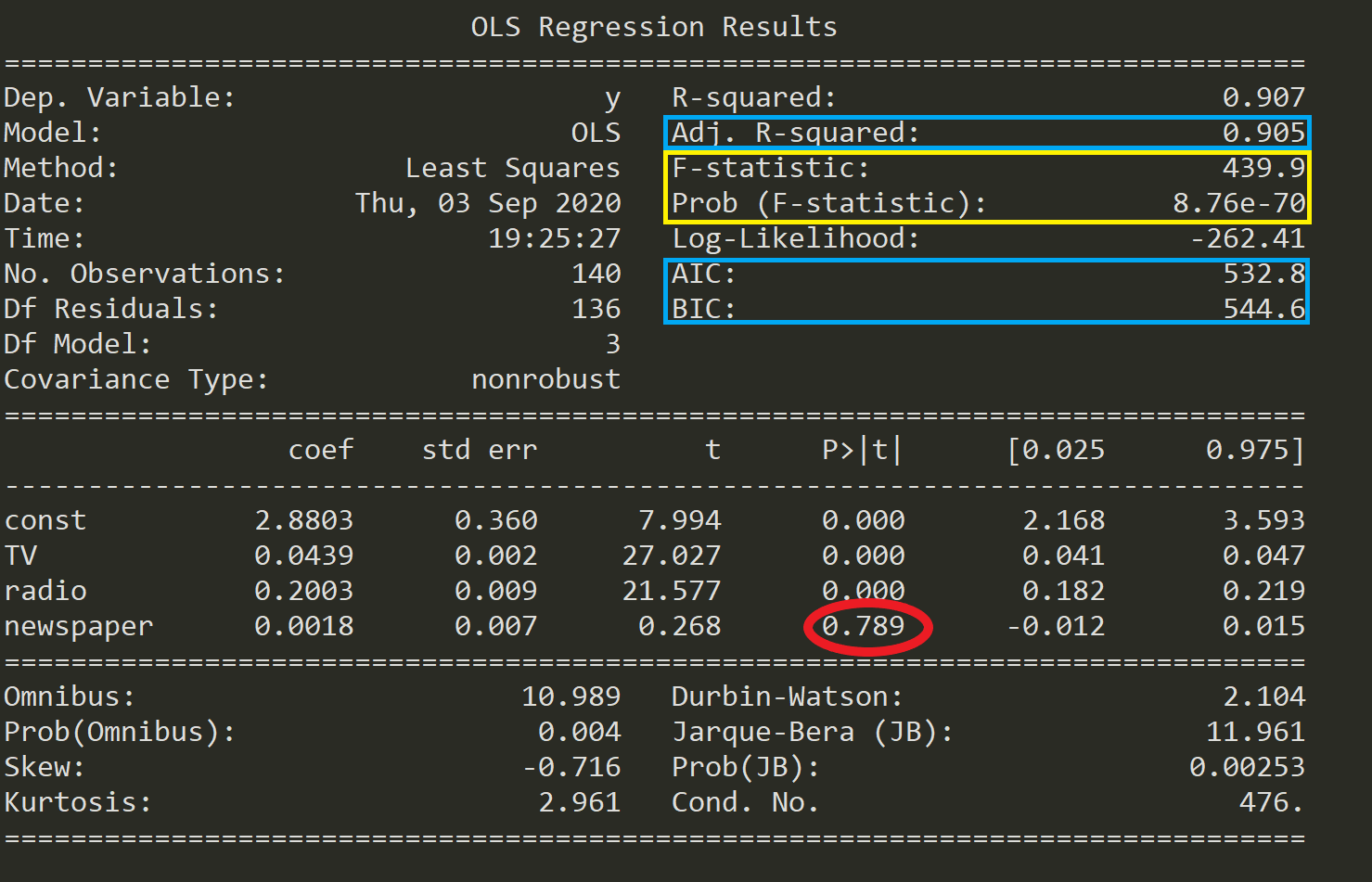Let’s see below what these numbers mean.
下面让我们看看这些数字的含义。
假设检验 (Hypothesis Test)
One of the fundamental questions you should answer while running a MLR model is, whether or not, at least one of the predictors is useful in predicting the output. What if the relationship between the independent variables and target is just by chance and there is no actual impact on sales due to any of the predictors?
运行MLR模型时，您应该回答的基本问题之一是， 至少有一个预测变量对预测输出有用 。 如果自变量与目标之间的关系仅仅是偶然的，并且由于任何预测因素而对销售没有实际影响，该怎么办？
We need to perform a Hypothesis Test to answer this question and check our assumptions. It all starts by forming a Null Hypothesis (H0), which states that all the coefficients are equal to zero, and there’s no relationship between predictors and target (meaning that a model with no independent variables fits the data as well as your model):
我们需要执行假设检验来回答这个问题并检查我们的假设。 这一切都始于形成一个零假设(H0) ，该假设指出所有系数都等于零，并且预测变量和目标变量之间没有关系(这意味着没有自变量的模型既适合数据又适合您的模型)：
Towards Data Science
迈向数据科学

On the other hand, we need to define an Alternative Hypothesis (Ha), which states that at least one of the coefficients is not zero, and there is a relationship between predictors and target (meaning that your model fits the data better than the intercept-only model):
另一方面，我们需要定义一个替代假设(Ha) ，该假设指出至少一个系数不为零，并且预测变量与目标之间存在关系(这意味着您的模型比截距更适合数据仅限型号)：
Towards Data Science
迈向数据科学

If we want to reject the Null Hypothesis and have confidence in our regression model, we need to find strong statistical evidence. To do this we perform a hypothesis test, for which we use the F-Statistic.
如果我们想拒绝零假设并对我们的回归模型有信心，我们需要找到有力的统计证据。 为此，我们执行假设检验，为此我们使用F统计量 。

If the value of F-statistic is equal to or very close to 1, then the results are in favor of the Null Hypothesis and we fail to reject it.
如果F统计量的值等于或非常接近1，则结果支持零假设，我们无法拒绝它。

As we can see in the table above (marked in yellow), the F-statistic is 439.9, thus providing strong evidence against the Null Hypothesis (that all coefficients are zero). Next, we also need to check the probability of occurrence of the F-statistic (also marked in yellow) under the assumption that the null hypothesis is true, which is 8.76e-70, an exceedingly small number lower than 1%. This means that there is much less than 1% probability that the F-statistic of 439.9 could have occurred by chance under the assumption of a valid Null hypothesis.
正如我们在上表中看到的(以黄色标记)，F统计量为439.9，因此有力地证明了零假设(所有系数均为零)。 接下来，我们还需要在原假设为真(8.76e-70)的情况下，检查F统计量 (也用黄色标记) 的发生概率 ，该数值非常小，低于1％。 这意味着在有效的Null假设的假设下，偶然发生F统计量439.9的可能性要小于1％。
Having said this, we can reject the Null Hypothesis and be confident that at least one predictor is useful in predicting the output.
话虽如此，我们可以否定零假设，并相信至少有一个预测变量可用于预测输出。
产生模型 (Generate models)
Running a Linear Regression model with many variables including irrelevant ones will lead to a needlessly complex model. Which of the predictors are important? Are all of them significant to our model? To find that out, we need to perform a process called feature selection. The 2 main methods for feature selection are:
运行包含许多不相关变量的线性回归模型将导致不必要的复杂模型。 哪些预测指标很重要？ 它们对我们的模型都重要吗？ 为了找出答案，我们需要执行一个称为功能选择的过程。   特征选择的2种主要方法是：
Forward Selection: where predictors are added one at a time beginning with the predictor with the highest correlation with the dependent variable. Then, variables of greater theoretical importance are incorporated to the model sequentially, until a stopping rule is reached. 正向选择：从与因变量相关性最高的预测变量开始，一次将预测变量添加一次。 然后，将具有较大理论重要性的变量顺序合并到模型中，直到达到停止规则为止。 Backward Elimination: where you start with all variables in the model, and remove the variables that have the least statistically significant (greater p-value), until a stopping rule is reached. 向后消除：从模型中的所有变量开始，然后删除统计上意义最小的变量(较大的p值)，直到达到停止规则为止。
Although both methods can be used, unless the number of predictors is larger than the sample size (or number of events), it’s usually preferred to use a backward elimination approach.
尽管两种方法都可以使用，但是除非预测变量的数量大于样本大小(或事件的数量)，否则通常首选使用向后消除方法。
You can find a full example and implementation of these methods in this link.
您可以在此链接中找到这些方法的完整示例和实现。
比较型号 (Compare models)
Every time you add an independent variable to a model, the R² increases, even if the independent variable is insignificant. In our model, are all predictors contributing to an increase in sales? And if so, are they all doing it in the same extent?
每当您向模型添加自变量时，即使自变量无关紧要，R²也会增加。 在我们的模型中，所有预测因素是否都有助于增加销售额？ 如果是这样，他们是否都在同一程度上做到这一点？
As opposed to R², Adjusted R² is a measure that increases only when the independent variable is significant and affects the dependent variable. So,if your R² score increases but the Adjusted R² score decreases as you add variables to the model, then you know that some features are not useful and you should remove them.
An interesting finding in the table above is that the p-value for newspaper is super high (0.789, marked in red). Finding the p-value for each coefficient will tell if the variable is statistically significant to predict the target or not.
上表中的一个有趣发现是报纸的p值超高(0.789，红色标记)。 查找每个系数的p值将说明该变量在统计上是否对预测目标有意义。

As a general rule of thumb, if the p-value for a given variable is less than 0.05 then there is a strong relationship between that variable and the target.
作为一般经验法则，如果给定变量的p值小于0.05，则该变量与目标之间存在很强的关系。

This way, including the variable newspaper doesn’t seem to be appropriate to reach a robust model, and removing it may improve the performance and generalization of the model.
这样，包括可变报纸似乎并不适合建立一个健壮的模型，删除它可以改善模型的性能和通用性。
Besides Adjusted R² score you can use other criteria to compare different regression models:
除了调整后的R²得分外，您还可以使用其他条件比较不同的回归模型：
Akaike Information Criterion (AIC): is a technique used to estimate the likelihood of a model to predict/estimate the future values. It rewards models that achieve a high goodness-of-fit score and penalizes them if they become overly complex. A good model is the one that has minimum AIC among all the other models. 赤池信息准则(AIC)：是一种用于估计模型预测/估计未来价值的可能性的技术。 它会奖励获得高拟合优度分数的模型，如果模型过于复杂，则会对其进行惩罚。 一个好的模型是所有其他模型中具有最小AIC的模型。 Bayesian Information Criterion (BIC): is another criteria for model selection that measures the trade-off between model fit and complexity, penalizing overly complex models even more than AIC. 贝叶斯信息准则(BIC)：是模型选择的另一个标准，它衡量模型拟合与复杂度之间的权衡，对过于复杂的模型造成的惩罚甚至超过了AIC。
假设条件 (Assumptions)
Because Linear Regression models are an approximation of the long-term sequence of any event, they require some assumptions to be made about the data they represent in order to remain appropriate. Most statistical tests rely upon certain assumptions about the variables used in the analysis, and when these assumptions are not met, the results may not be trustworthy (e.g. resulting in Type I or Type II errors).
由于线性回归模型是任何事件的长期序列的近似值，因此需要对它们表示的数据进行一些假设才能保持适当。 大多数统计检验都依赖于有关分析中使用的变量的某些假设，如果不满足这些假设，则结果可能不可信(例如，导致I型或II型错误)。
Linear Regression models are linear in the sense that the output is a linear combination of the input variables, and only suited for modeling linearly separable data. Linear Regression models work under various assumptions that must be present in order to produce a proper estimation and not to depend solely on accuracy scores:
从输出是输入变量的线性组合的意义上讲，线性回归模型是线性的，并且仅适用于对线性可分离数据进行建模。 线性回归模型在各种假设下工作，这些假设必须存在才能产生适当的估计，而不仅仅是依赖于准确性得分：
Linearity: the relationship between the features and target must be linear. One way to check the linear relationships is to visually inspect scatter plots for linearity. If the relationship displayed in the scatter plot is not linear, then we’d need to run a non-linear regression or transform the data. 线性 ：特征与目标之间的关系必须是线性的。 检查线性关系的一种方法是目视检查散点图的线性。 如果散点图中显示的关系不是线性的，那么我们需要运行非线性回归或转换数据。 Homoscedasticity: the variance of the residual must be the same for any value of x. Multiple linear regression assumes that the amount of error in the residuals is similar at each point of the linear model. This scenario is known as homoscedasticity. Scatter plots are a good way to check whether the data are homoscedastic, and also several tests exist to validate the assumption numerically (e.g. Goldfeld-Quandt, Breusch-Pagan, White) 均方差：对于任何x值，残差的方差必须相同。 多元线性回归假设残差的误差量在线性模型的每个点都相似。 这种情况称为同调。 散点图是检查数据是否为同方差的好方法，并且还存在一些测试以数值验证该假设(例如，Goldfeld-Quandt，Breusch-Pagan，White)
Towards Data Science
迈向数据科学

No multicollinearity: data should not show multicollinearity, which occurs when the independent variables (explanatory variables) are highly correlated to one another. If this happens, there will be problems in figuring out the specific variable that contributes to the variance in the dependent/target variable. This assumption can be tested with the Variance Inflation Factor (VIF) method, or through a correlation matrix. Alternatives to solve this issue may be centering the data (deducting the mean score), or conducting a factor analysis and rotating the factors to insure independence of the factors in the linear regression analysis. 无多重共线性：数据不应显示多重共线性，当自变量(解释变量)彼此高度相关时，就会发生多重共线性。 如果发生这种情况，将很难找出导致因变量/目标变量差异的特定变量。 可以使用方差通货膨胀系数(VIF)方法或通过相关矩阵来检验此假设。 解决此问题的替代方法可能是将数据居中(扣除平均得分)，或进行因子分析并旋转因子以确保线性回归分析中因子的独立性。 No autocorrelation: the value of the residuals should be independent of one another. The presence of correlation in residuals drastically reduces model’s accuracy. If the error terms are correlated, the estimated standard errors tend to underestimate the true standard error. To test for this assumption, you can use the Durbin-Watson statistic. 无自相关 ：残差的值应彼此独立。 残差中存在相关性会大大降低模型的准确性。 如果误差项相关，则估计的标准误差往往会低估真实的标准误差。 要测试此假设，可以使用Durbin-Watson统计信息。 Normality of residuals: residuals must be normally distributed. Normality can be checked with a goodness of fit test (e.g. Kolmogorov-Smirnov or Shapiro-Wilk tests), and if data is not normally distributed, a non-linear transformation (e.g. log transformation) might fix the issue. 残差的正态性 ：残差必须正态分布。 可以使用拟合优度检验(例如Kolmogorov-Smirnov或Shapiro-Wilk检验)来检查正态性，如果数据不是正态分布的，则非线性转换(例如对数转换)可以解决此问题。
Towards Data Science
迈向数据科学

Assumptions are critical because if they are not valid, then the analytical process can be considered unreliable, unpredictable, and out of control. Failing to meet the assumptions can lead to draw conclusions that are not valid or scientifically unsupported by the data.
假设是至关重要的，因为如果假设无效，那么分析过程将被认为是不可靠，不可预测且不受控制的。 不符合这些假设可能导致得出无效的结论或数据在科学上不支持的结论。
You can find a full testing of the assumptions in this link.
您可以在此链接中找到对假设的完整测试。
最后的想法 (Final thoughts)
Although MLR models extend the scope of SLR models, they are still linear models, meaning that the terms included in the model are incapable of showing any non-linear relationships between each other or representing any sort of non-linear trend. You should also be careful when predicting a point outside the observed range of features since the relationship among variables may change as you move outside the observed range (a fact that you can’t know because you don’t have the data).
尽管MLR模型扩展了SLR模型的范围，但它们仍然是线性模型，这意味着模型中包含的术语无法显示彼此之间的任何非线性关系或表示任何种类的非线性趋势。 在预测要素的观察范围之外的点时，您还应该小心，因为变量之间的关系可能会随着您移出观察范围而改变(这是您不知道的原因，因为您没有数据)。

The observed relationship may be locally linear, but there may be unobserved non-linear relationships on the outside range of your data.
观察到的关系可能是局部线性的，但是在数据的外部范围上可能存在未观察到的非线性关系。

Linear models can also model curvatures by including non-linear variables such as polynomials and transforming exponential functions. The linear regression equation is linear in the parameters, meaning you can raise an independent variable by an exponent to fit a curve, and still remain in the “linear world”. Linear Regression models can contain log terms and inverse terms to follow different kinds of curves and yet continue to be linear in the parameters.
线性模型还可以通过包含非线性变量(例如多项式)和变换指数函数来对曲率建模 。 线性回归方程的 参数是线性的 ，这意味着您可以通过指数增加自变量以拟合曲线，但仍保留在“线性世界”中。 线性回归模型可以包含对数项和逆项，以遵循不同类型的曲线，但参数仍保持线性。
While the independent variable is squared, the model is still linear in the parameters

Regressions like Polynomial Regression can model non-linear relationships, and while a linear equation has one basic form, non-linear equations can take many different forms. The reason you might consider using Non-linear Regression Models is that, while linear regression can model curves, it might not be able to model the specific curve that exists in your data.
像多项式回归这样的回归可以对非线性关系进行建模，虽然线性方程式具有一种基本形式，但是非线性方程式可以采用许多不同形式。 您可能会考虑使用非线性回归模型的原因是，尽管线性回归可以对曲线进行建模，但它可能无法对数据中存在的特定曲线进行建模。
You should also know that OLS is not the only method to fit your Linear Regression model, and other optimization methods like Gradient Descent are more adequate to fit large datasets. Applying OLS to complex and non-linear algorithms might not be scalable, and Gradient Descent can be computationally cheaper (faster) for finding the solution. Gradient Descent is an algorithm that minimizes functions, and given a function defined by a set of parameters, the algorithm starts with an initial set of parameter values and iteratively moves toward a set of parameter values that minimize the function. This iterative minimization is achieved using derivatives, taking steps in the negative direction of the function gradient.
Towards Data Science
迈向数据科学

Another key thing to take into account is that outliers can have a dramatic effect on regression lines and the correlation coefficient. In order to identify them it’s essential to perform Exploratory Data Analysis (EDA), examining the data to detect unusual observations, since they can impact the results of our analysis and statistical modeling in a drastic way. In case you recognize any, outliers can be imputed (e.g. with mean / median / mode), capped (replacing those outside certain limits), or replaced by missing values and predicted.
要考虑的另一项关键是， 离群值会对回归线和相关系数产生巨大影响 。 为了识别它们，执行探索性数据分析(EDA) ，检查数据以检测异常观察非常重要，因为它们会以极大的方式影响我们的分析和统计建模的结果。 如果您识别出任何异常，则可以对异常值进行估算(例如，使用均值/中位数/众数)，设置上限(替换超出某些限制的那些值)或替换为缺失值并进行预测。
Finally, some limitations of Linear Regression models are:
最后， 线性回归模型的一些局限性是：
Omitted variables. It is necessary to have a good theoretical model to suggest variables that explain the dependent variable. In the case of a simple two-variable regression, one has to think of other factors that might explain the dependent variable, since there may be other “unobserved” variables that explain the output. 省略的变量 。 必须有一个良好的理论模型来提出解释因变量的变量。 在简单的二变量回归的情况下，必须考虑可能解释因变量的其他因素，因为可能会有其他“无法观察的”变量解释输出。 Reverse causality. Many theoretical models predict bidirectional causality — that is, a dependent variable can cause changes in one or more explanatory variables. For instance, higher earnings may enable people to invest more in their own education, which, in turn, raises their earnings. This complicates the way regressions should be estimated, calling for special techniques. 反向因果关系 。 许多理论模型都预测双向因果关系，即因变量可能导致一个或多个解释变量发生变化。 例如，更高的收入可能使人们能够对自己的教育进行更多的投资，从而增加了他们的收入。 这使估计回归的方式变得复杂，需要特殊的技术。 Mismeasurement. Factors might be measured incorrectly. For example, aptitude is difficult to measure, and there are well-known problems with IQ tests. As a result, the regression using IQ might not properly control for aptitude, leading to inaccurate or biased correlations between variables like education and earnings. 测量错误 。 可能会错误地评估因素。 例如，能力很难测量，并且智商测试存在众所周知的问题。 结果，使用智商的回归可能无法适当地控制才能，导致教育和收入等变量之间的关系不准确或有偏见。 Too limited a focus. A regression coefficient provides information only about how small changes — not large changes — in one variable relate to changes in another. It will show how a small change in education is likely to affect earnings but it will not allow the researcher to generalize about the effect of large changes. If everyone became college educated at the same time, a newly minted college graduate would be unlikely to earn a great deal more because the total supply of college graduates would have increased dramatically. 焦点太有限了 。 回归系数仅提供有关一个变量中的微小变化(而不是大变化)与另一变量中的变化之间的关系的信息。 它会显示出教育的微小变化可能会如何影响收入，但不会使研究人员对较大变化的影响进行概括。 如果每个人都同时接受大学教育，那么刚毕业的大学毕业生就不太可能赚更多的钱，因为大学毕业生的总供应量将大大增加。

翻译自: https://towardsdatascience.com/your-guide-to-linear-regression-models-df1d847185db

一般线性模型和线性回归模型

展开全文机器学习 python 人工智能 深度学习
• 在谈及广义线性模型是什么之前，我想先分析一下线性回归模型有什么限制。在这里先说明一点，以下分析的线性回归模型，是考虑了随机误差项的完整的线性回归模型。 首先我想说明一下关于响应变量（y）的分布，以及响应...
在谈及广义线性模型是什么之前，我想先分析一下线性回归模型有什么限制。在这里先说明一点，以下分析的线性回归模型，是考虑了随机误差项的完整的线性回归模型。
首先我想说明一下关于响应变量（y）的分布，以及响应变量和解释变量（x）之间的关系的区别。在线性回归中，往往我们会认为响应变量服从正态分布（且方差不变）、响应变量和解释变量之间服从线性关系，这时候我们使用线性回归才能得到好的效果，那么我们应该怎么理解这两者呢，首先来看一下图：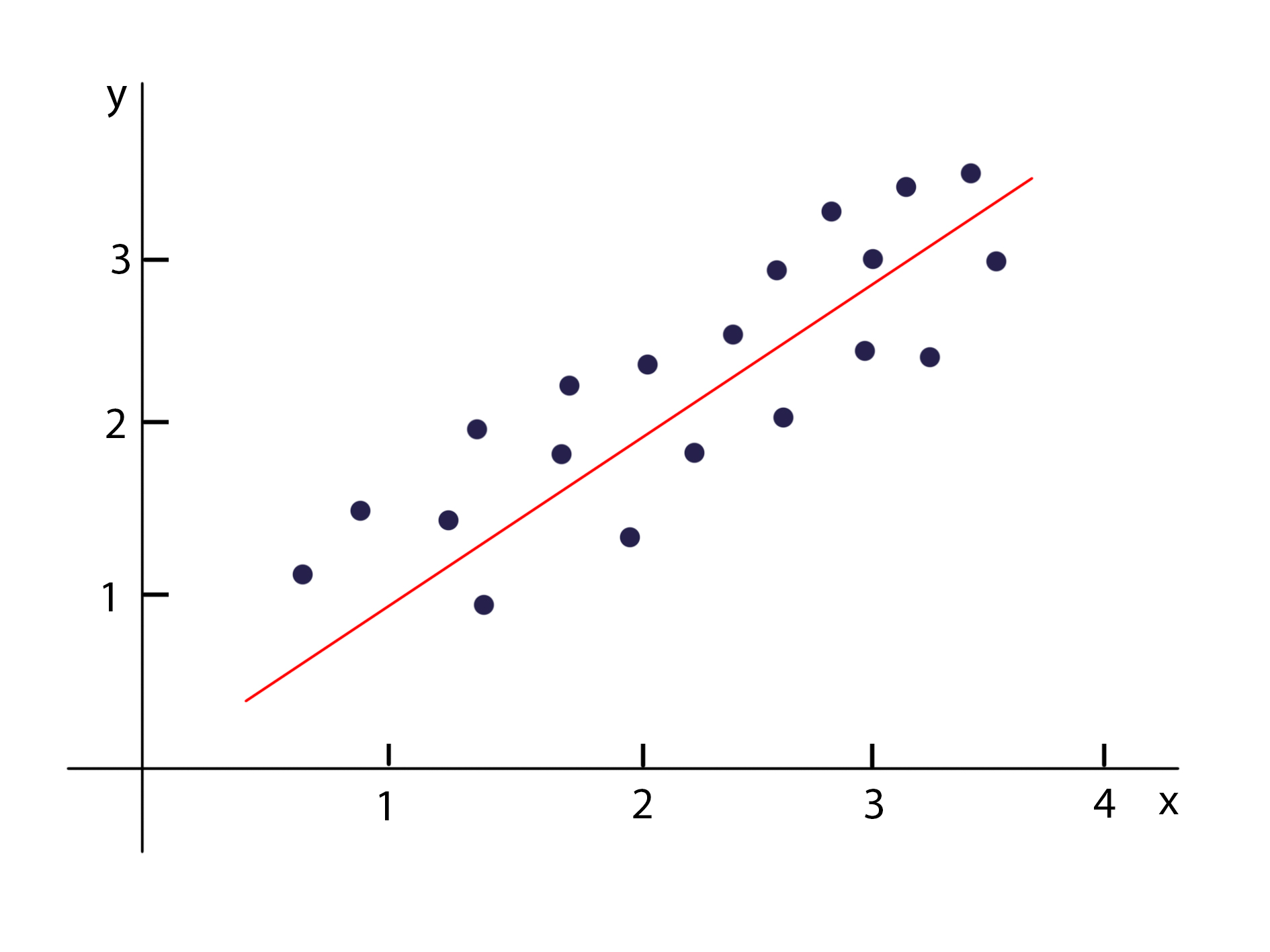这是一个很经典的线性分布的数据，可以看到随着x增大y也增大，而且两个的关系能够用一条直线近似表达，所以我们说x和y之间有线性关系。如果我们只看y的数据，也就是说把这个二维图降维到只有y轴：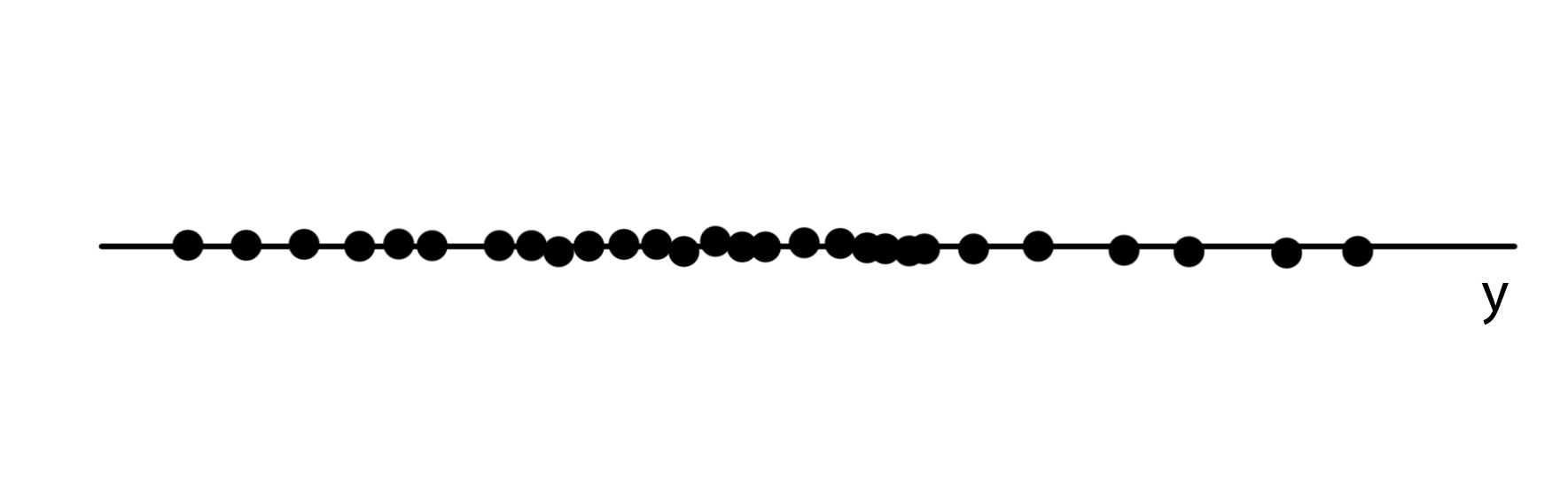上图就相当于把最初的图的点投影到y轴上，这时候没有了x，反映的就是y的数据的分布状况。
明白了什么是响应变量的分布之后，就开始正式讨论线性回归的局限性。首先，正如一开始说的，线性回归的响应变量需要服从正态分布，这是基于高斯-马尔可夫定理得到的：在线性回归模型中，如果误差满足零均值、同方差且互不相关，则回归系数的最佳线性无偏估计就是普通最小二乘法估计。或者简单来说，这时候使用最小二乘法的线性回归的估计效果最好。
要说清楚这个结论，还是从头推导一次比较好。首先，我们还记得线性回归模型可以表达为：

Y

i

=

a

0

+

a

1

X

i

+

e

i

Y_i = a_0 + a_1 X_i + e_i

a_0、a_1是非随机但不可观察的参数，X_i是非随机且可观察的一般变量，e_i是不可观察的随机变量，因此Yi是可观察的随机变量。对于一个把随机误差也考虑的完整的线性回归模型，模型的输出并不等于回归方程的输出，还需要加上随机误差项。响应变量因为考虑了随机误差而被看作随机变量。我们基于数据拟合一个线性回归模型，其实只是求出a_0、a_1这两个参数，主要是基于最小化损失函数（最小二乘法）得到的：

L

o

s

s

=

∑

(

a

0

+

a

1

X

i

−

Y

i

′

)

2

Loss = \sum (a_0 + a_1 X_i - Y_i')^2

其中，Y_i’是真实数据（观察数据），因为模型考虑了随机误差，所以这里可以假设，观察数据等于我们训练完成后的线性回归模型的输出，所以，当我们最小化了损失函数，我们就可以得到：

L

o

s

s

=

∑

(

(

a

0

+

a

1

X

i

)

−

(

a

0

′

+

a

1

′

X

i

+

e

i

)

)

2

Loss = \sum ((a_0 + a_1 X_i) - (a_0' + a_1' X_i + e_i))^2

L

o

s

s

=

∑

(

e

i

)

2

Loss = \sum (e_i)^2

以上的式子说明了，即使响应变量和解释变量确实服从线性关系，即使模型已经训练完成，因为随机误差的存在，损失函数也不可能等于0。在这里我们可以得到一个结论，对于一个训练完成的线性回归模型，它的损失函数和随机误差项是相关的。
接下来我们再看另一个例子，现在我们基于数据做线性回归得到一个模型：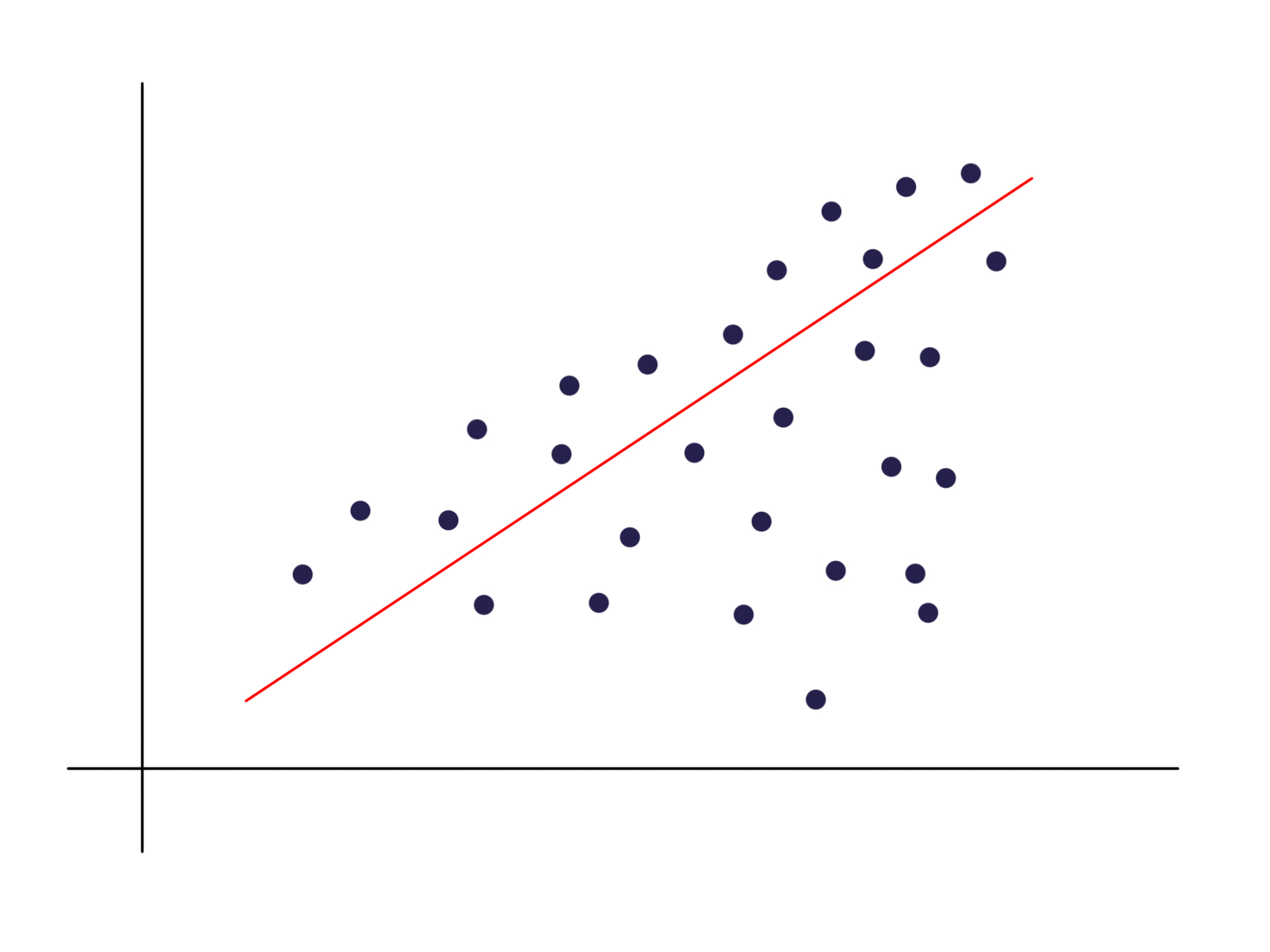在分位数回归中也提到这种数据分布，分布会随着x的增大越来越分散，也就是说方差变大，虽然基于线性回归的算法我们也依然可以得到一条回归直线，但不能说回归效果很好，因为它并没有反映出数据越来越分散的特征，所以我们才需要分位数回归。那么这种数据分布和随机误差项有什么联系呢，一开始提到随机误差项表征数据因随机误差无法完美落在回归曲线上，假如我们基于这个数据集和我们得到的线性模型，针对两个小范围的x的数据，画出随机误差项的分布，就可以得到：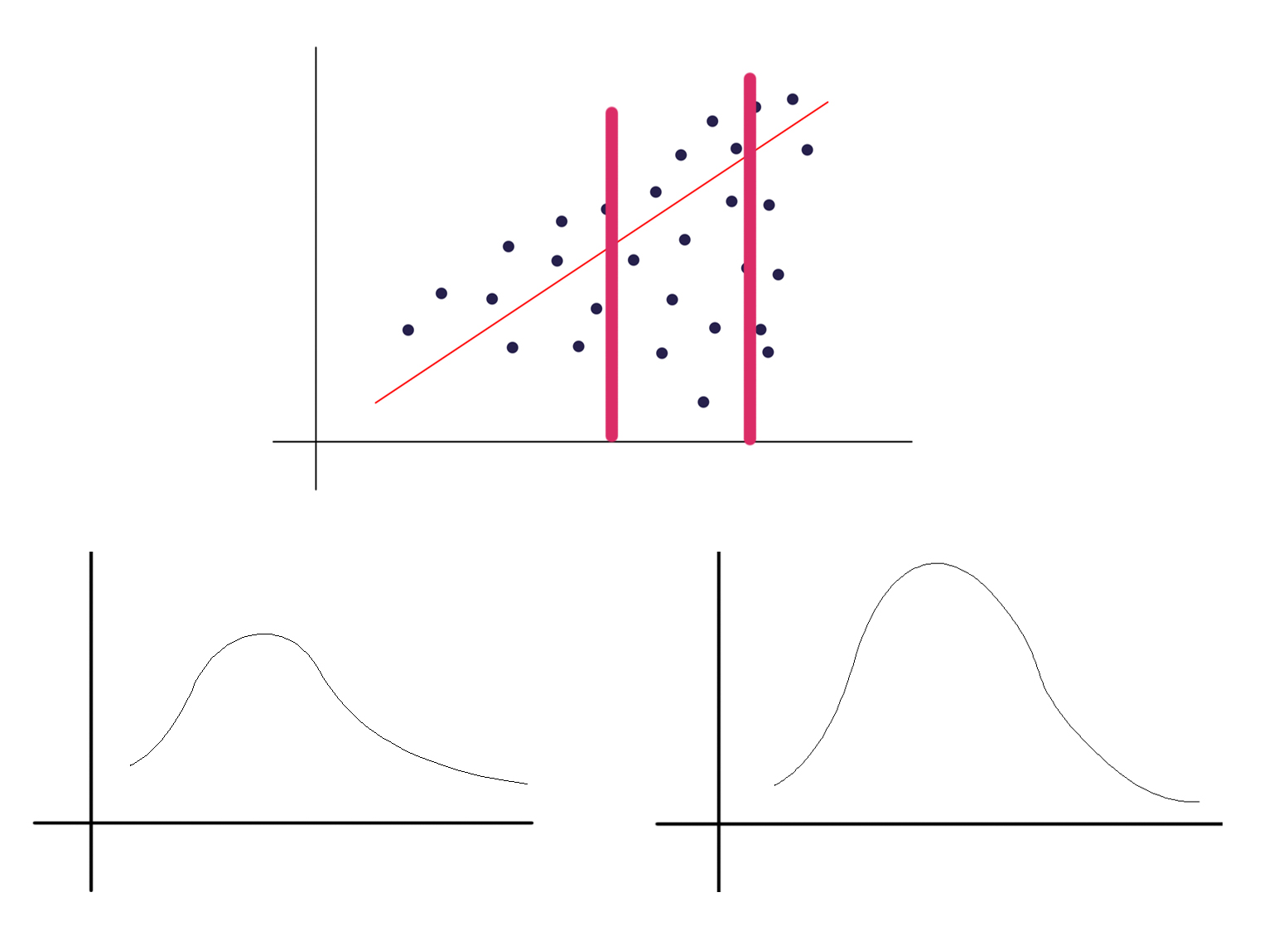上图大概画出了两个范围的随机误差的分布状况（它们的均值都为0），可以看出，第一，随机误差的分布不是正态分布，第二，随机误差的分布的方差不是同一个数值。那么我们再回想一下，对于一开始提到的理想的数据分布，应该是数据对称地分布在回归曲线两侧，同时分散程度是接近的，这也就意味着，随机误差项服从正态分布，且独立同分布。
经过上面的论述，我们就知道随机误差项服从正态分布时模型的效果最好，而因为响应变量的随机性主要受到随机误差的影响，所以可以认为响应变量也服从正态分布：

y

i

∼

N

(

a

0

+

a

1

X

i

,

σ

2

)

y_i \sim N(a_0 + a_1 X_i, \sigma^2)

其中，期望等于回归函数的输出（假设随机误差的期望为0），方差等于随机误差的方差
除此之外，我们还需要注意，在我们进行线性回归的时候，其实一开始就假设了解释变量和响应变量之间是服从线性关系的，假设他们之间不服从线性分布（以上图的数据分布为例），而我们依然采用线性回归，那么他们的残差就被模型认为是随机误差造成的，这也就导致了随机误差不服从正态分布。再回想一下，其实响应变量因为随机误差的存在才被认为是随机分布的，当随机误差不服从正态分布，响应变量也就不服从正态分布了。
上面的结论也说明了，虽然响应变量的分布和响应变量解释变量之间的关系是两回事，但是它们之间是相互联系的。
上面主要分析了线性回归要求响应变量服从正态分布才能有好的回归效果，这是第一个限制，第二个是要求响应变量和解释变量之间是线性关系，因为这两个限制条件，就导致了线性回归模型在有些情况下是不适用的，于是就有了我们的广义线性模型，它主要改进了线性回归的两个限制：不要求响应变量服从正态分布，以及响应变量和解释变量之间可以服从非线性关系。

想浏览更多关于数学、机器学习、深度学习的内容，可浏览本人博客

展开全文广义线性模型
• 基本线性回归和局部加权线性回归、岭回归、前向逐步回归 in Python
• 临床上，因变量和临床的...既往教程中我们介绍了使用R语言在COX回归模型基础上绘制限制立方条图，后台有不少粉丝说道不会制作logistic回归和线性回归限制立方条图，我们今天分别来讲讲。 先讲logistic回归绘制立方大数据
• 线性回归介绍及分别使用最小二乘法和梯度下降法对线性回归C++实现
• ## 多元线性回归的基础理解

万次阅读 多人点赞 2018-11-19 01:09:28
多元线性回归  Multivarate Linear Regression Multiple Feature 前面我们学习了一元线性回归，也动手亲自从底层编写了梯度下降算法来实现一元线性回归。相信大家已经对梯度下降和线性回归有了很清晰的理解了。 ...机器学习 梯度下降 正规方程 特征缩放
• 本文转自viewcode的《对线性回归、逻辑回归、各种回归的学习》，原文链接：点击打开链接 ...1. 线性回归 假设 特征 和 结果 都满足线性。即不大于一次方。这个是针对 收集的数据而言。 收集的数据中，每一个分量优化
• 对非齐次约束线性回归模型的狭义条件根方估计和广义条件根方估计进行讨论．利用相对效率定义比较两种根方估计的效率，证明在一定条件下，广义条件根方估计的效率不低于狭义条件根方估计，在根方参数的限制下比较了...
• 线性回归模型是一种比较简单的、容易解释的回归预测方法。在实际应用中，可以用线性回归模型简单判断特征与目标值之间是否存在某种线性相关的关系。若存在，可以用多个线性模型去表示回归值；若不存在，即可以考虑...
• 一、什么是线性回归 要理解这个名词含义那么就要分别理解什么是线性和回归： 线性：变量之间的关系是一次函数关系，如y=x+1那么x和y的关系就是线性关系。相反中x与y的关系就是非线性。 回归：最简单的理...机器学习 数据挖掘
• 机器学习线性回归学习心得Data science with the kind of power it gives you to analyze each and every bit of data you have at your disposal, to make smart & intelligent business decisions, is ...机器学习 python 人工智能 深度学习
• 线性回归（Linear Regression）对于二维数据而言，线性回归就是找出一个一次函数去拟合数据，使得平方误差最小。是的，这里的损失函数是平方损失。平方误差可以写做：∑im=(yi−xTiw)2\sum^m_i=(y_i-x_i^Tw)机器学习
• 1 主成分分析PCA2 主成分回归PCR3、偏最小二乘PLS4、岭回归Ridge regression参考： 1. PCA回归实现myself； 2. 多元线性回归 文库；...3. 多元线性回归中多重共线性问题的解决办法探讨_张凤莲.caj
• 线性回归——岭回归、lasso、前向逐步回归1.岭回归2.lasso3.前向逐步回归 接上一篇文章线性回归——局部加权线性回归，我们知道如何解决欠拟合，现在我们介绍一下当出现过拟合时我们怎么解决。 解决过拟合我们可以...lasso
• 线性回归 岭回归（Ridge） Lasso回归 线性回归 线性回归模型方程形式： 矩阵形式： 线性回归的任务就是要构造一个预测函数来映射，输入的特征矩阵和标签的线性关系。这个预测函数的本质就是我们需要构建...最小二乘法
• 线性回归原理，实现，应用。线性回归应该是机器学习最基本的问题了。它是利用称为线性回归方程的最小平方函数对一个或多个自变量和因变量之间关系进行建模的一种回归分析，这种函数是一个或多个称为回归系数的模型...python实现 ViF 异方差 梯度下降
• 回归问题的条件/前提： 1） 收集的数据。 2） 假设的模型，即一个...(3)cost function：评价线性回归是否拟合训练集的方法 (4)梯度下降：解决线性回归的方法之一 (5)feature scaling：加快梯度下降执行速度的方法机器学习算法
• ## 线性回归和逻辑回归的区别

万次阅读 多人点赞 2018-10-30 10:36:02
1. 线性回归 假设 特征 和 结果 都满足线性。即不大于一次方。这个是针对 收集的数据而言。 收集的数据中，每一个分量，就可以看做一个特征数据。每个特征至少对应一个未知的参数。这样就形成了一个线性模型函数，...
• 目录(?)  [+] 回归问题的条件/前提： 1） 收集的数据 2） 假设的模型，即一个...1. 线性回归 假设 特征 和 结果 都满足线性。即不大于一次方。这个是针对 收集的数据而言。 收集的数据中，每一个分量，就可以机器学习 逻辑回归
• 比如我们根据根据一家企业的运营质量、盈利、运营时间等来对企业进行估值，这属于估值问题，如果存在线性关系，属于线性回归问题。再比如我们对验证码图片进行识别，将图片通过线性函数也产生了一列值，但是我们的...python 机器学习 数据挖掘
• 我们在对多元线性回归的损失函数求导，并得出求解系数的式子和过程，在最后一步中我们需要左乘的逆矩阵，而逆矩阵存在的充分必要条件是特征矩阵不存在多重共线性。 首先解释一下逆矩阵存在的充分必要条件：也就是...
• 初级算法梳理——线性回归目录1.机器学习基本概念2.线性回归原理3.线性回归损失函数，代价函数，目标函数4.优化方法5.线性回归的评价指标6.sklearn参数详解1.机器学习基本概念2.线性回归的原理3.线性回归损失函数、...
• 在统计学中，线性回归方程是利用最小二乘函数对一个或多个自变量和因变量之间关系进行建模的一种回归分析。这种函数是一个或多个称为回归系数的模型参数的线性组合。只有一个自变量的情况称为简单回归，大于一个自...机器学习 算法
• Content线性回归的多重共线性1. 前提2. 由损失函数推导ω(基于最小二乘法OLS)3. 上述计算结果不成立3.1 多重共线性的机器学习解释3.2 多重共线性的解决4. Ridge & Lasso4.1 Ridge4.2 Lasso 线性回归的多重共线性...机器学习 矩阵
• 线性回归、逻辑回归、损失函数     回归问题的条件/前提： 1） 收集的数据 2） 假设的模型，即一个函数，这个函数里含有未知的参数，通过学习，可以估计出参数。然后利用这个模型...
• 多变量线性回归算法思想与单变量线性回归算法思想基本一致，（1）先找到损失函数，（2）求损失函数最小化后的参数；本文还简单介绍了特征缩放，正则方程以及矩阵不可逆的情况。（备注：图片都来自andrew老师的视频......